# Maharashtra State HSC Board Question Paper for Class 12th Maths 2017 In PDF

## MSBSHSE HSC Board 12th Class Question Paper Mathematics English Medium 2017 with Solutions – Free Download

Maharashtra State Board Class 12 Maths 2017 question paper with solutions are available on this page, by BYJU’S, in downloadable pdf format and also in the text, so that the students can prepare efficiently for the upcoming board exams. Maths Question Paper 2017 Class 12 is also uploaded here for reference. Students are able to access all the Maharashtra HSC previous year maths question papers. By solving these papers, students can get a clear idea of the question paper pattern and also the weightage of each of the chapters.

### MSBSHSE Class 12th Maths Question Paper With Solutions 2017

#### QUESTION PAPER CODE J – 541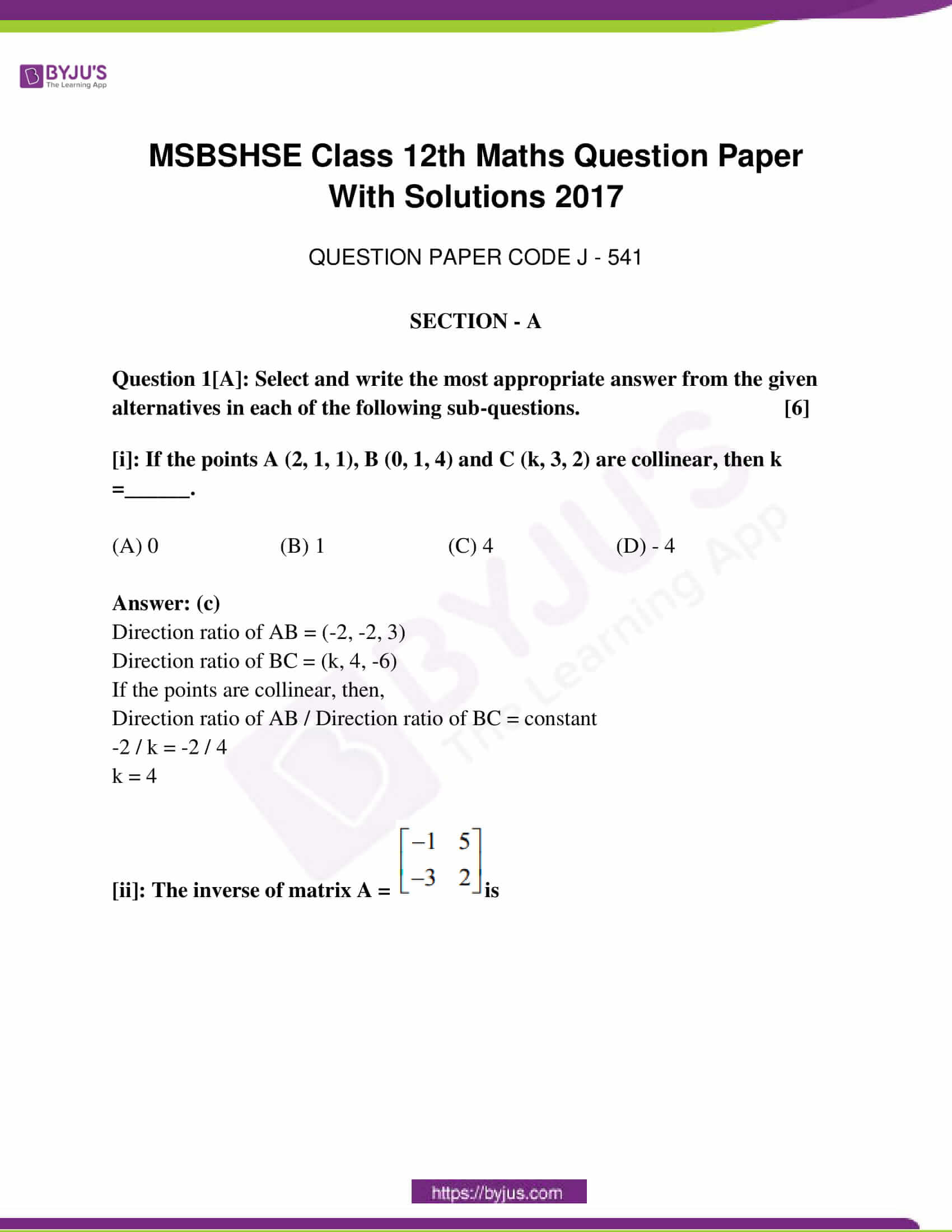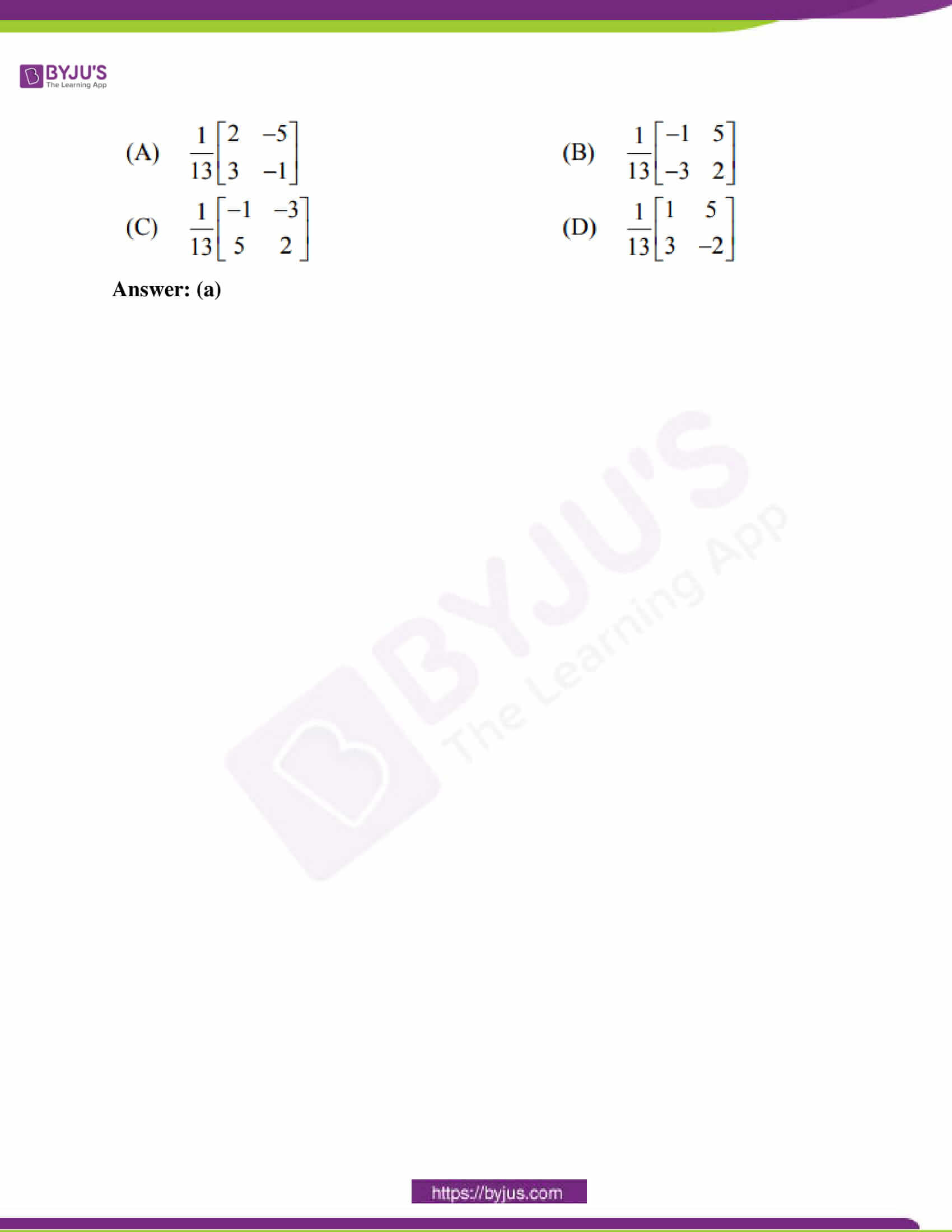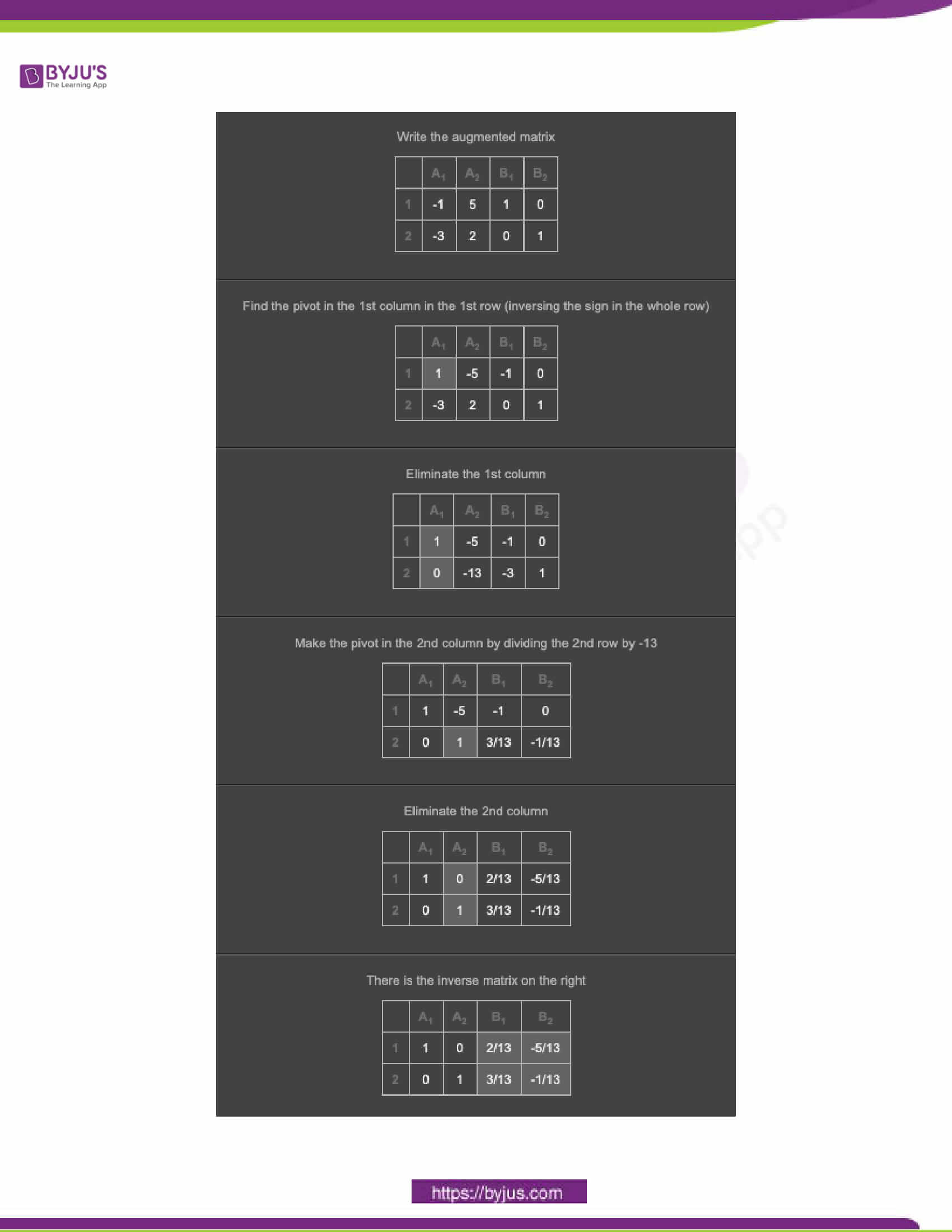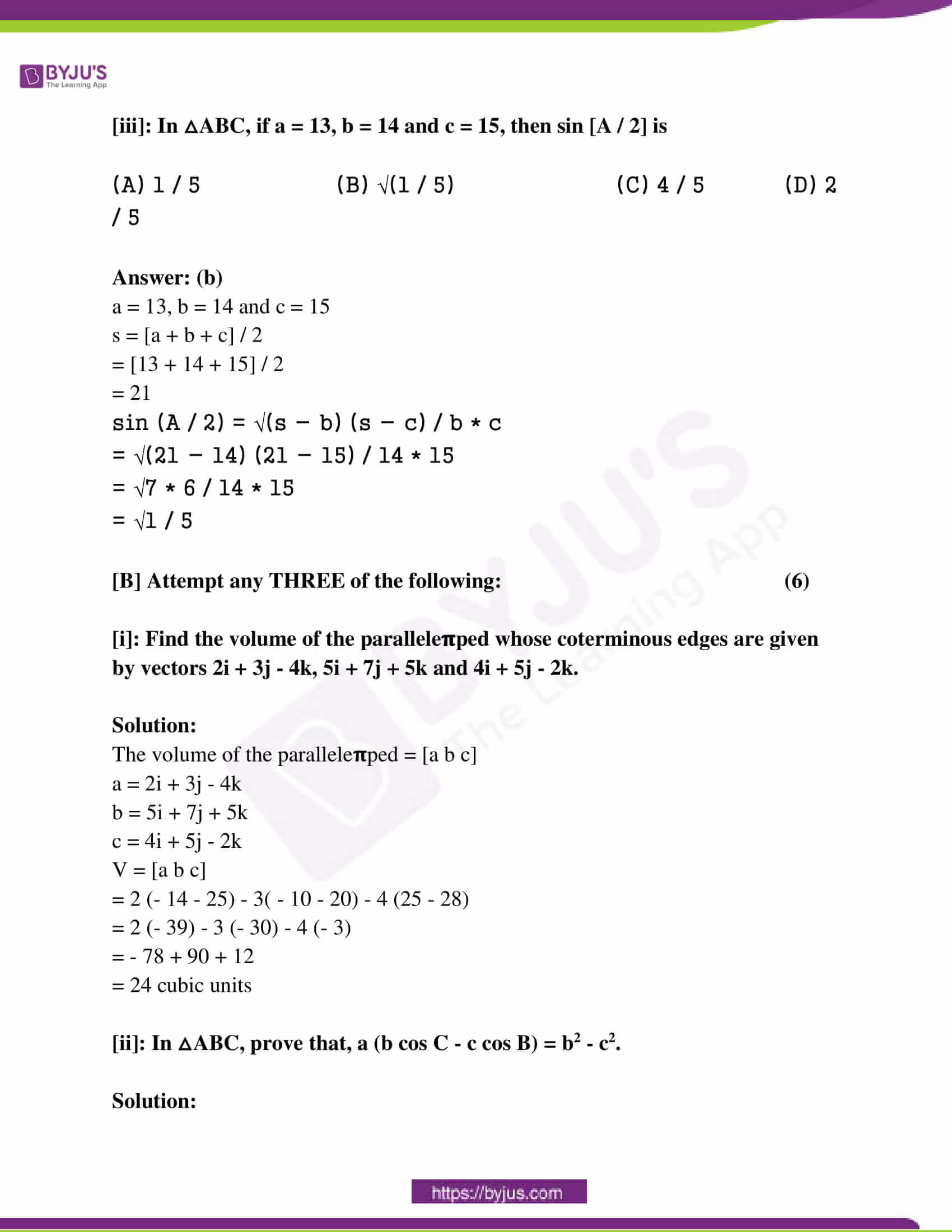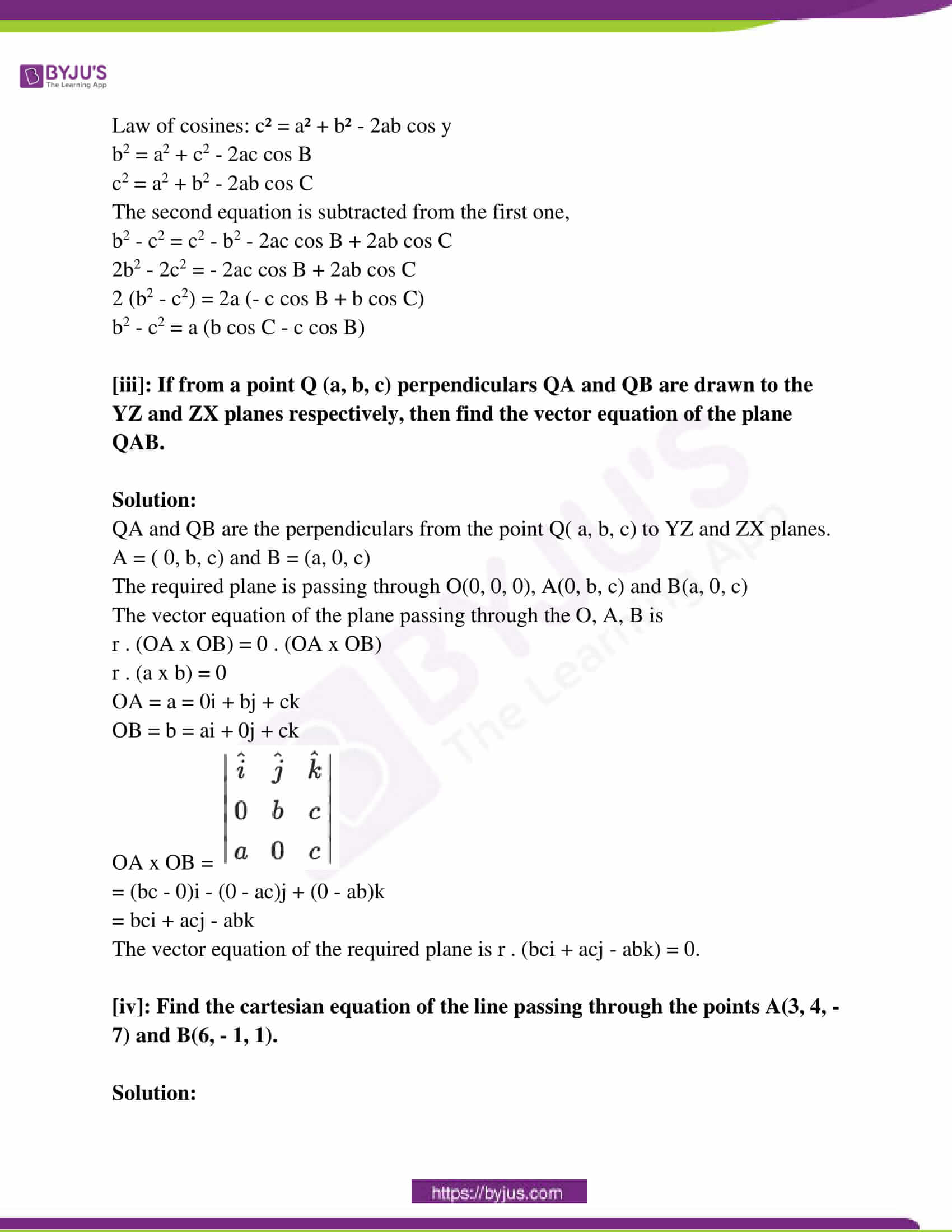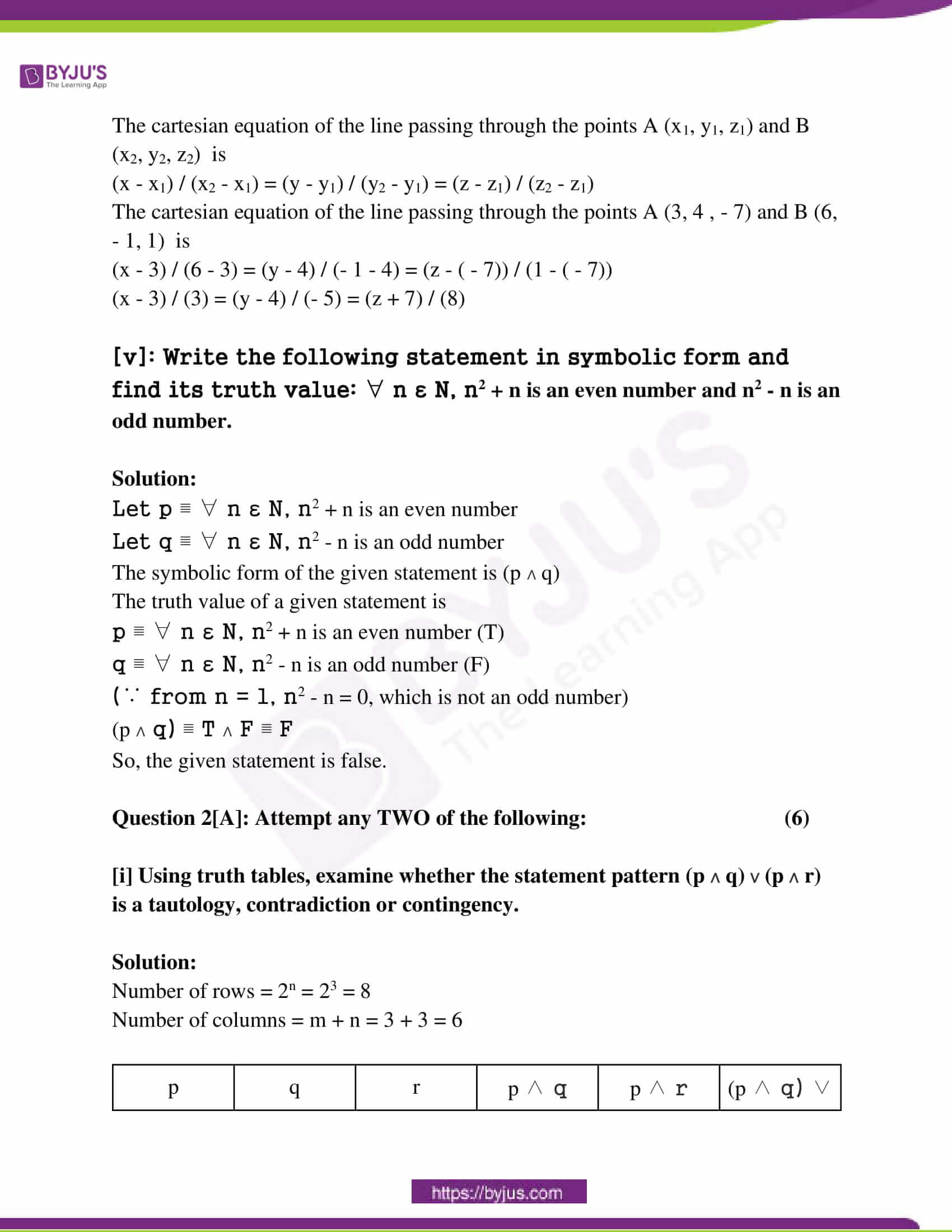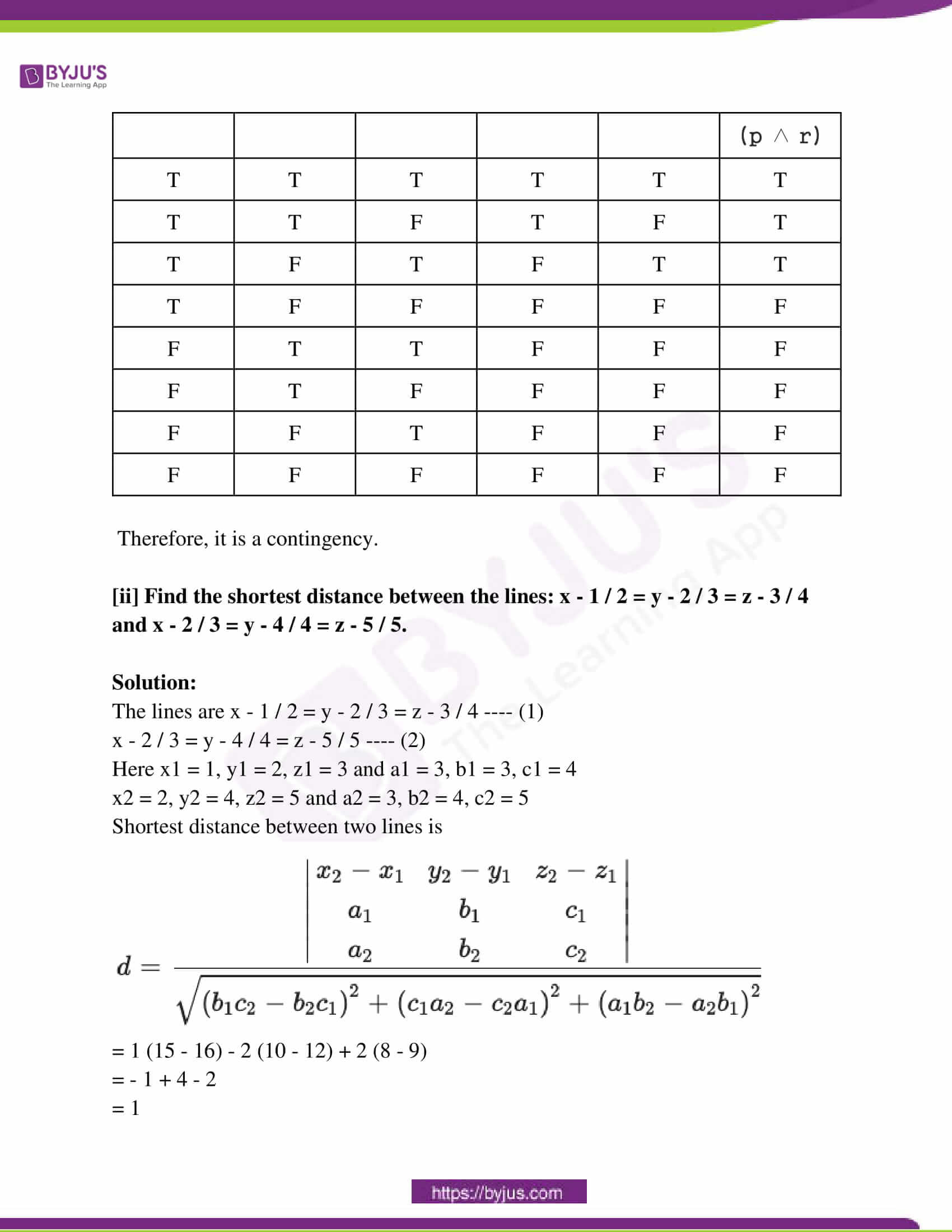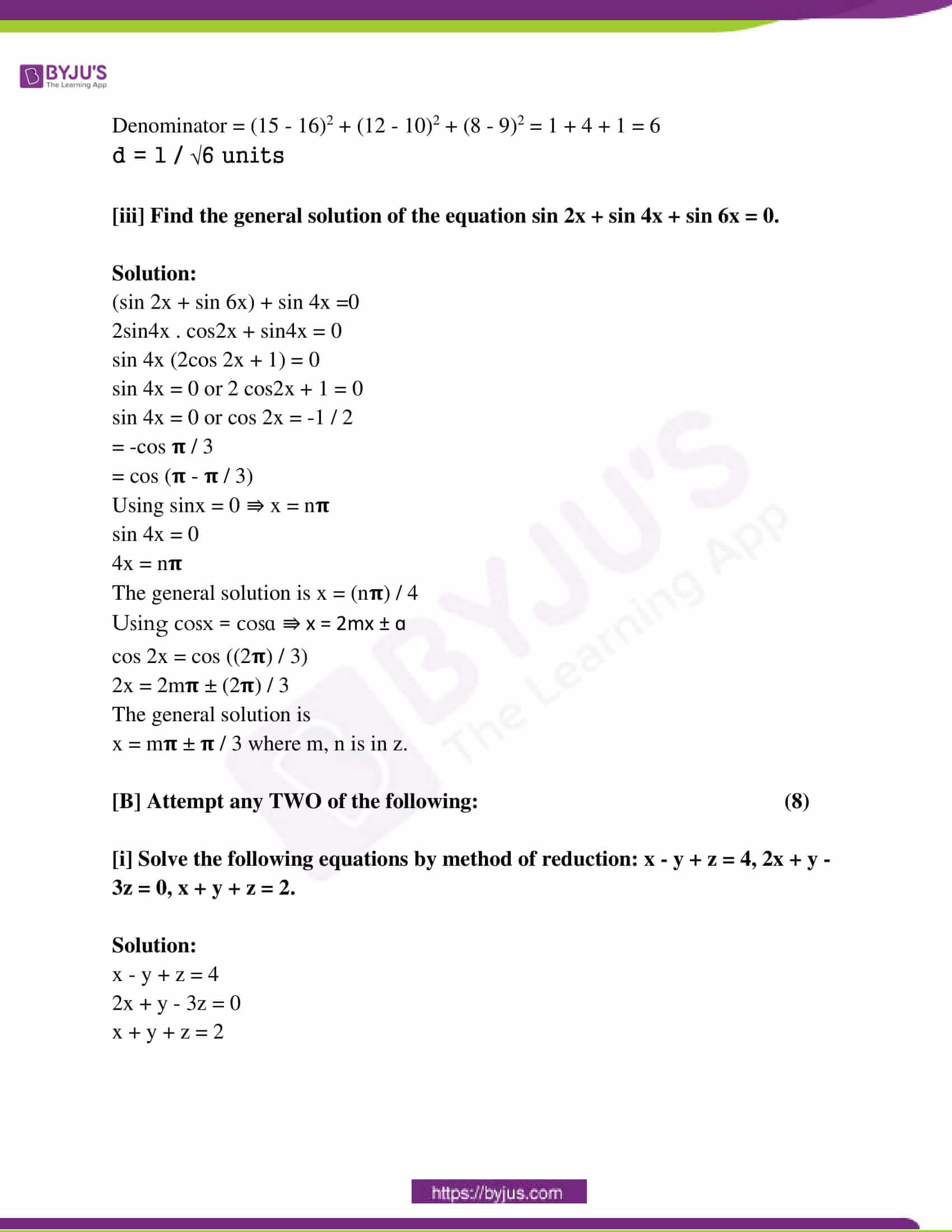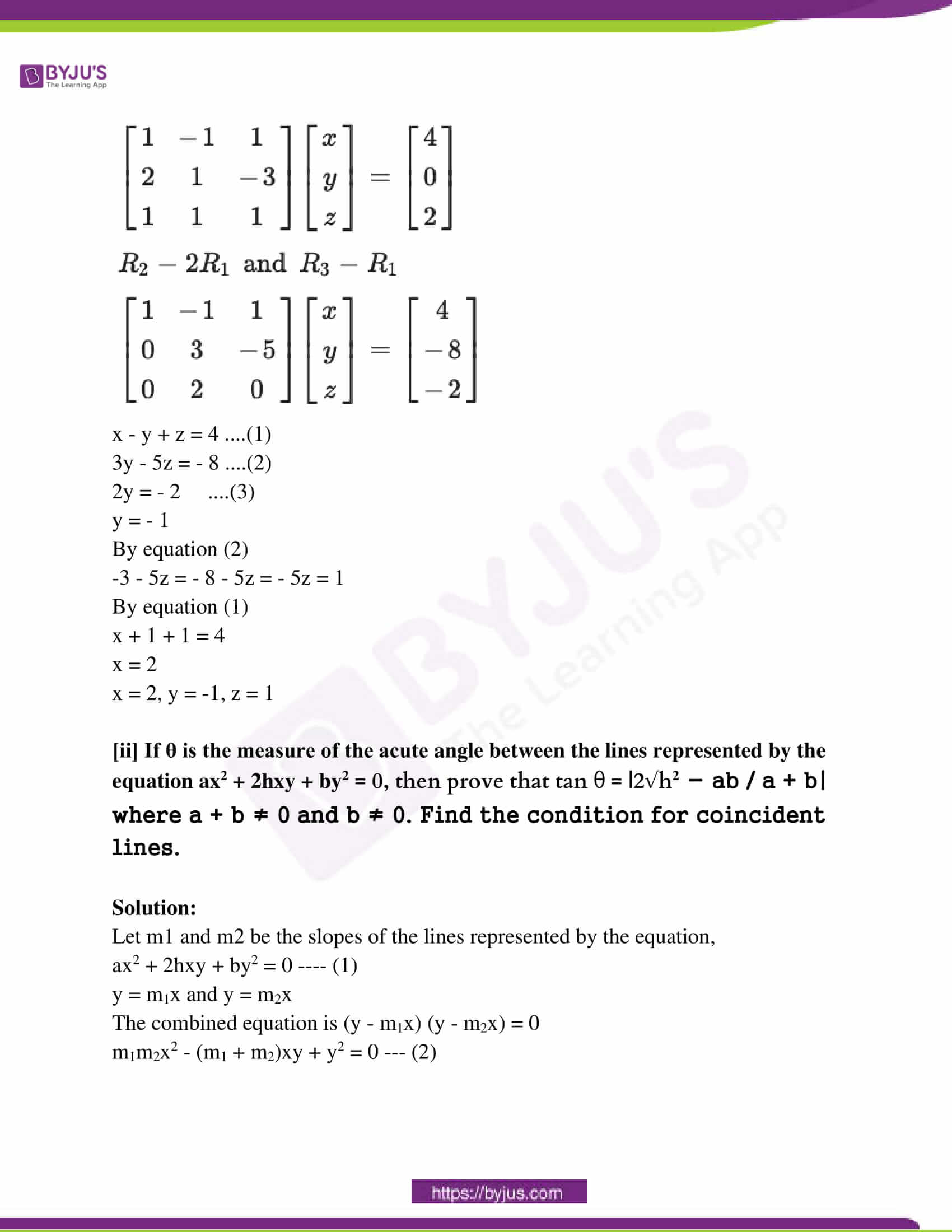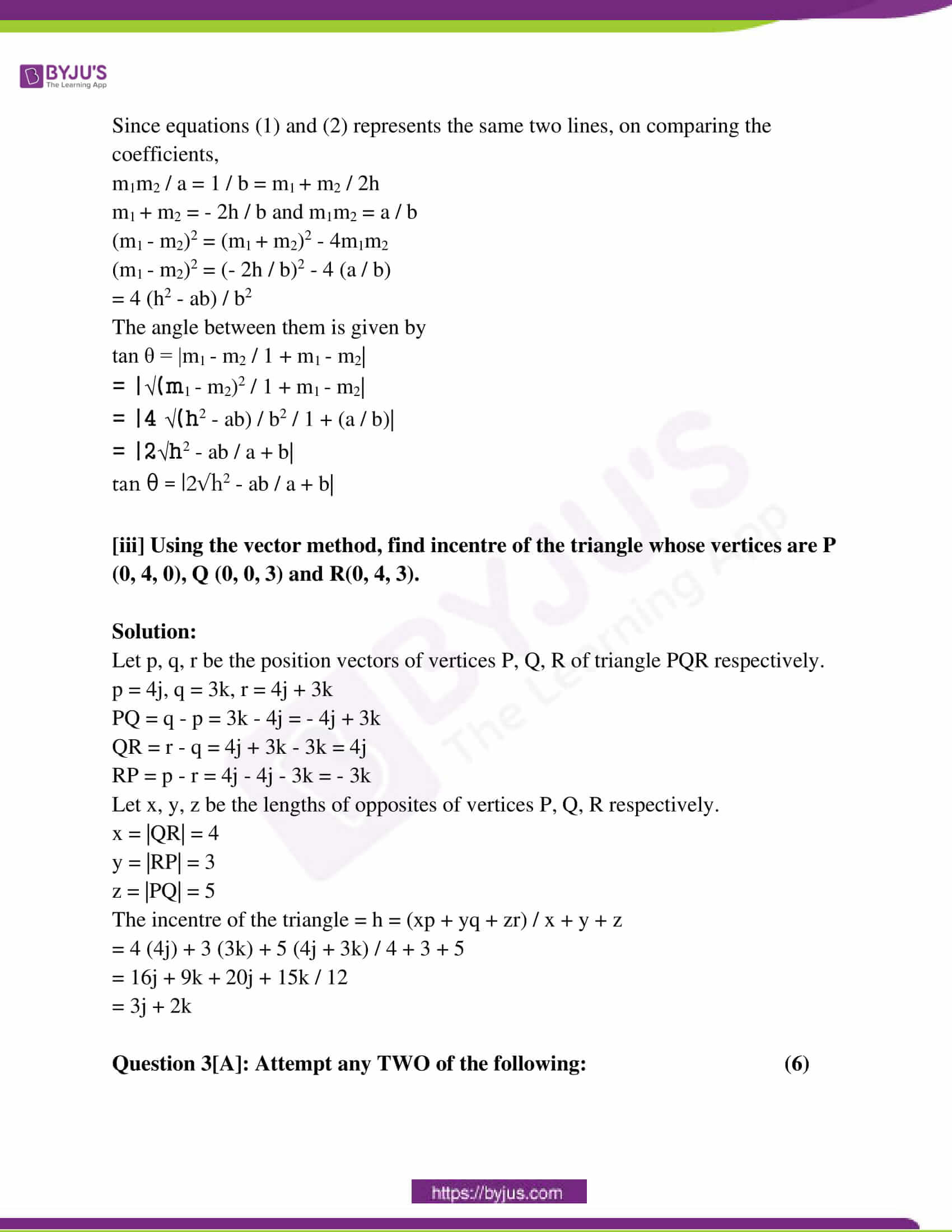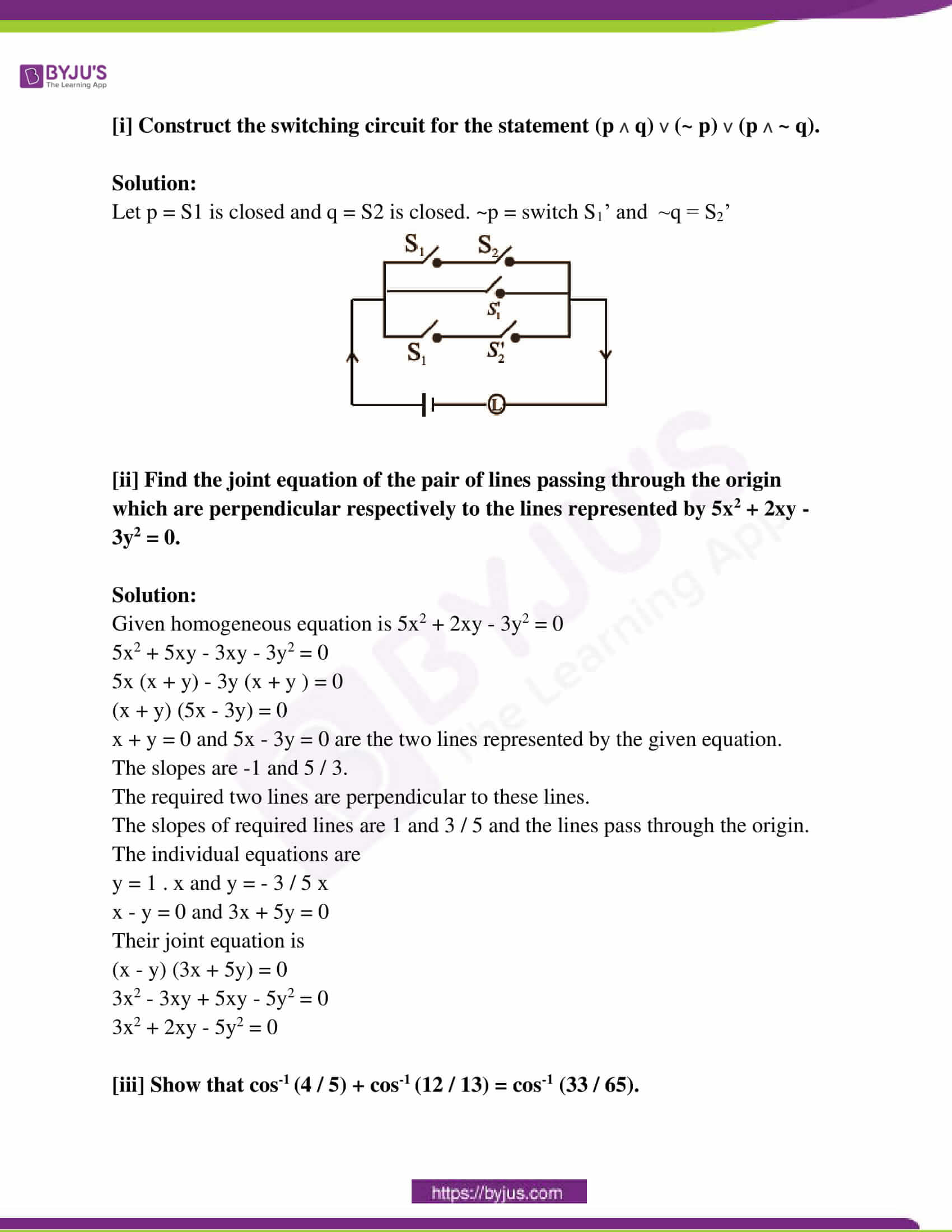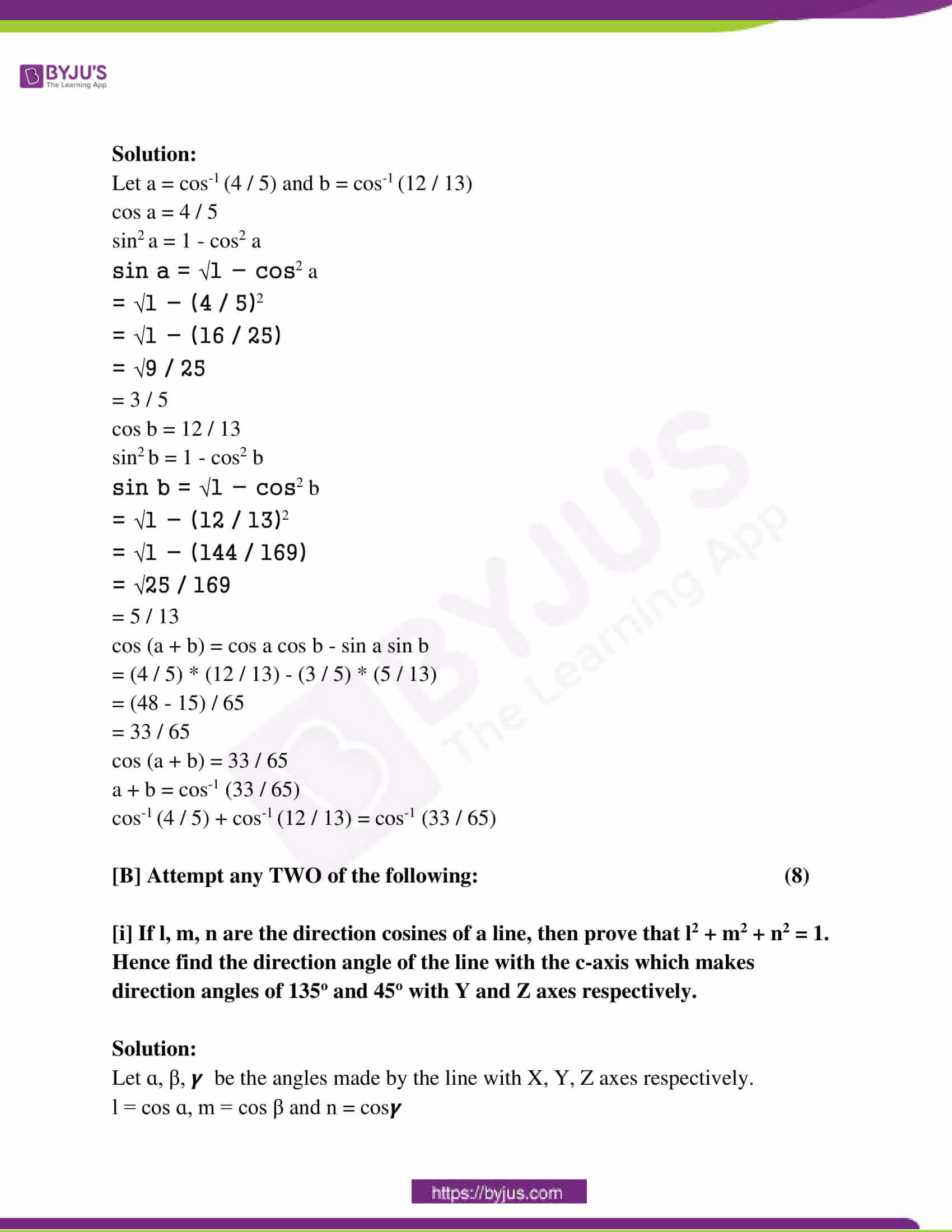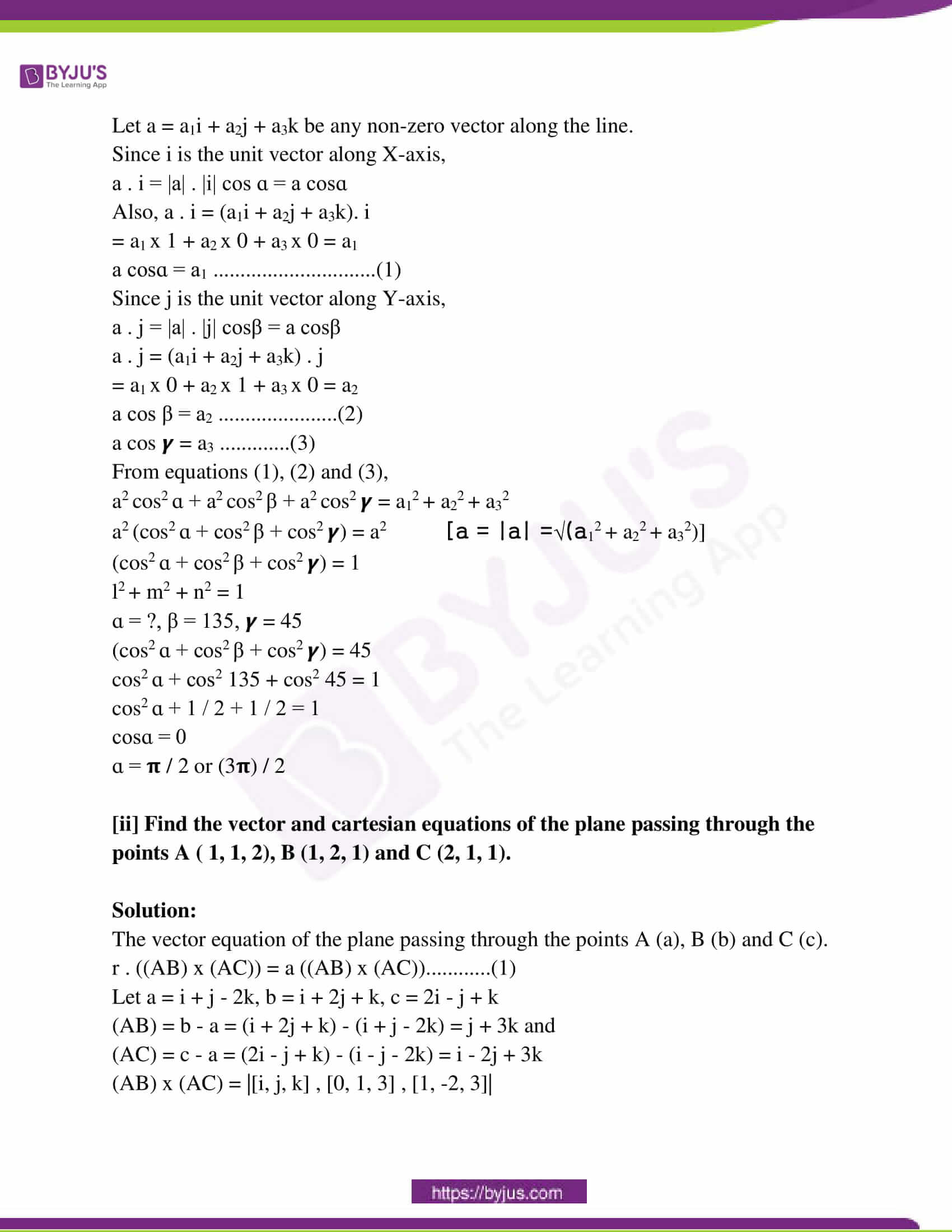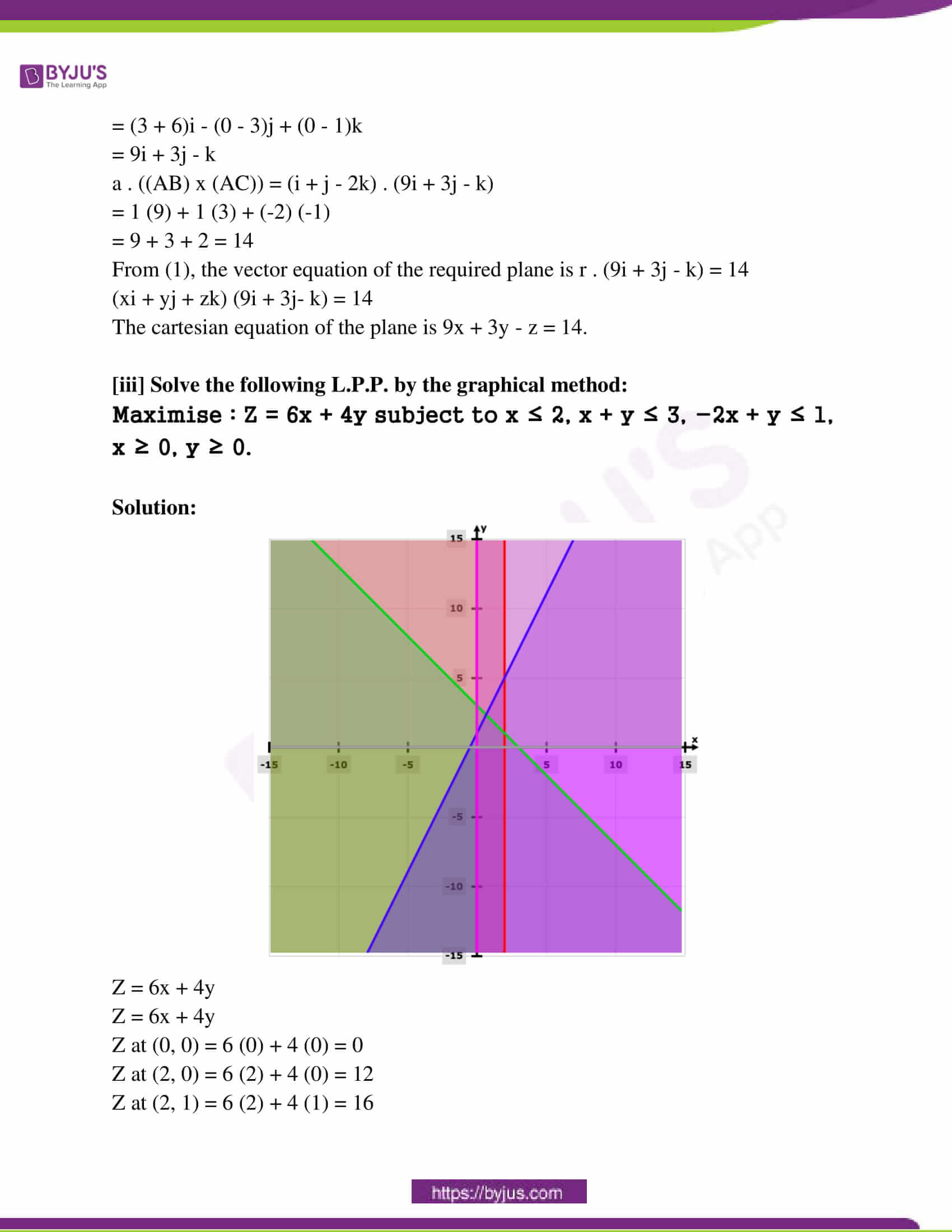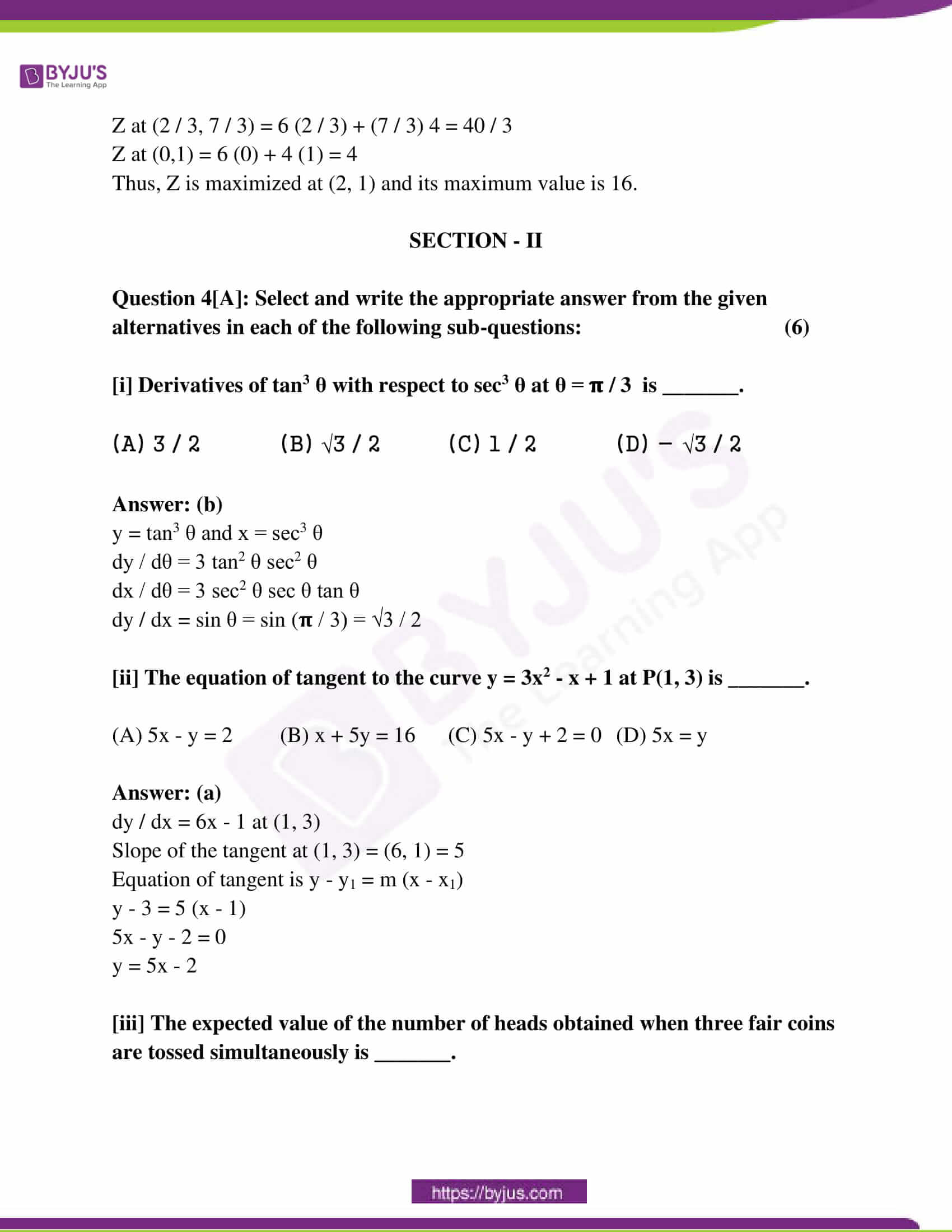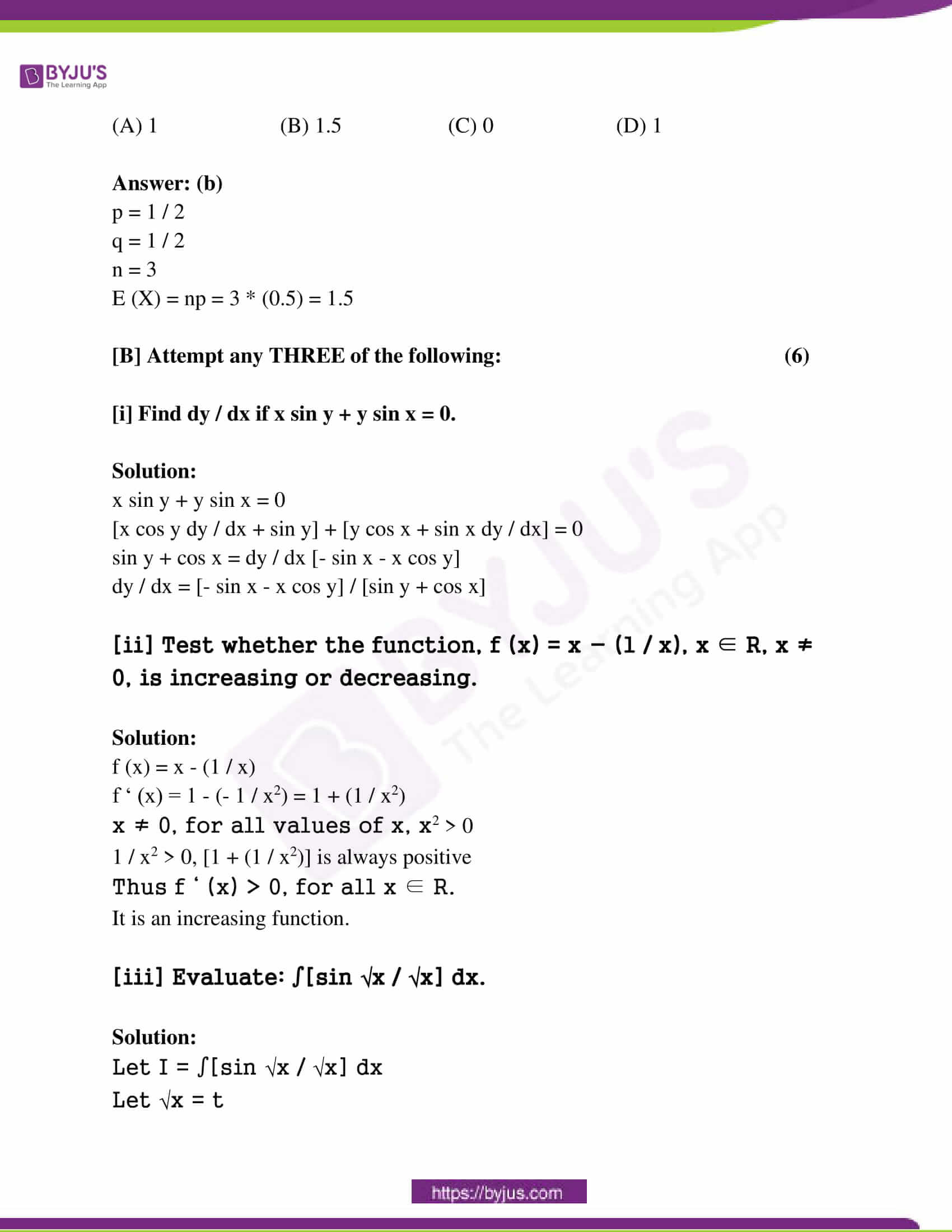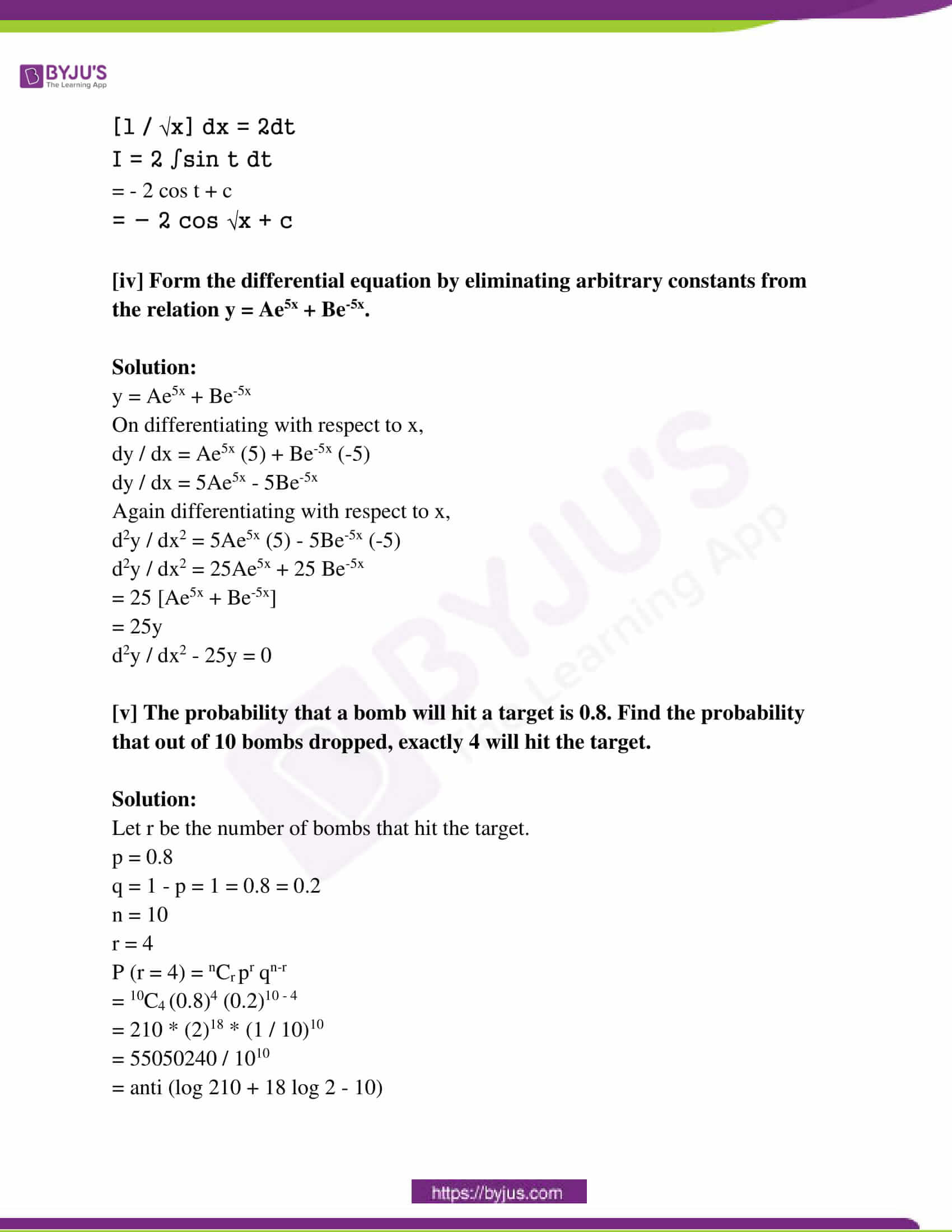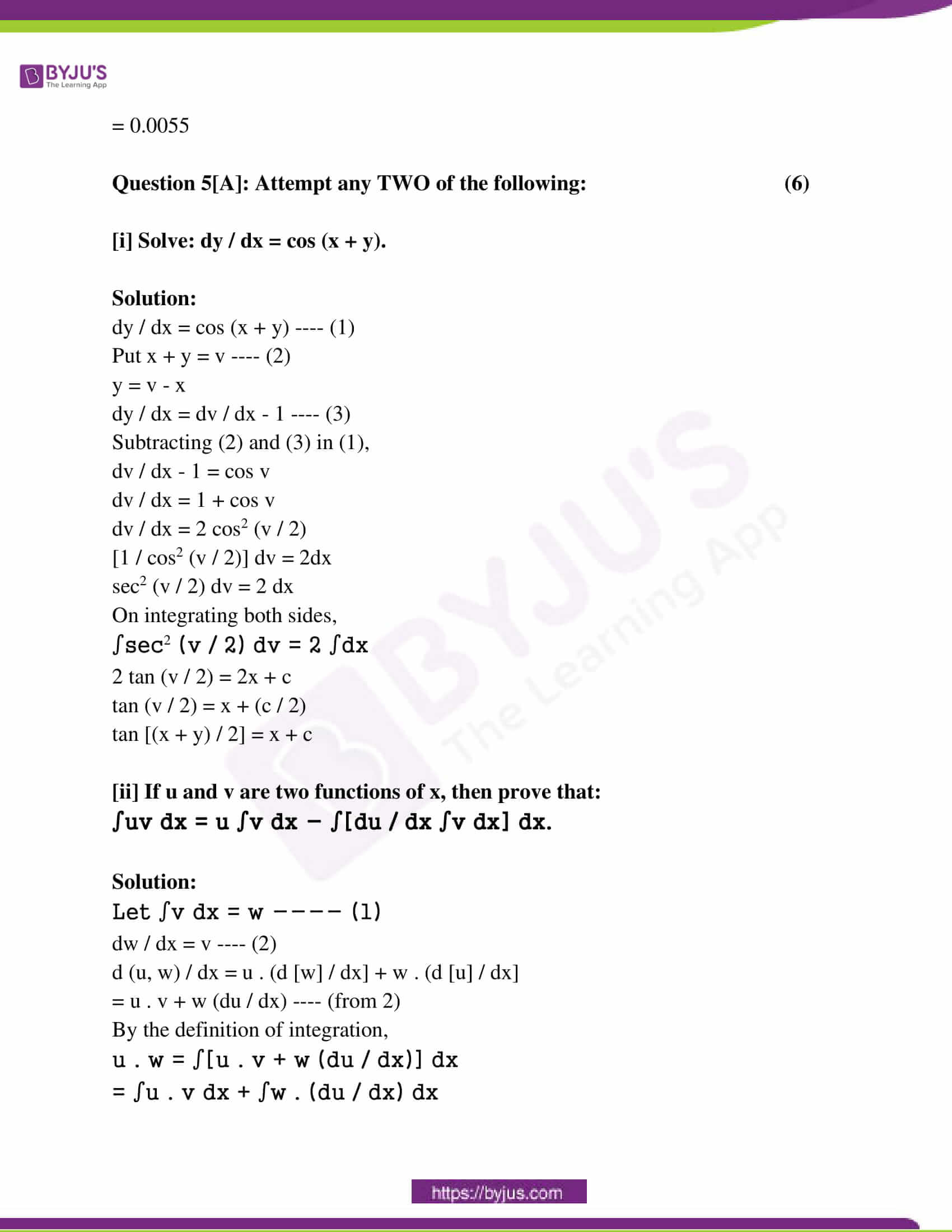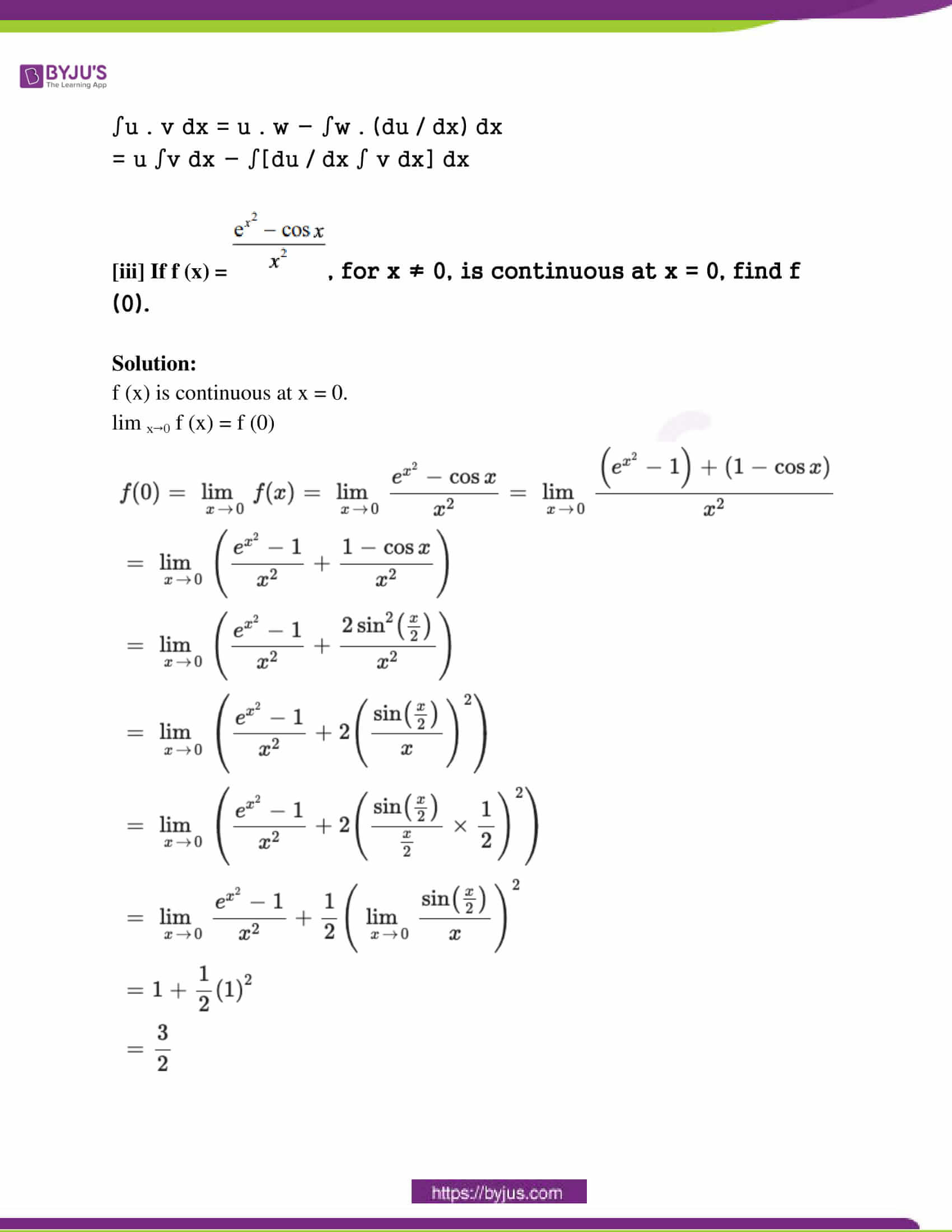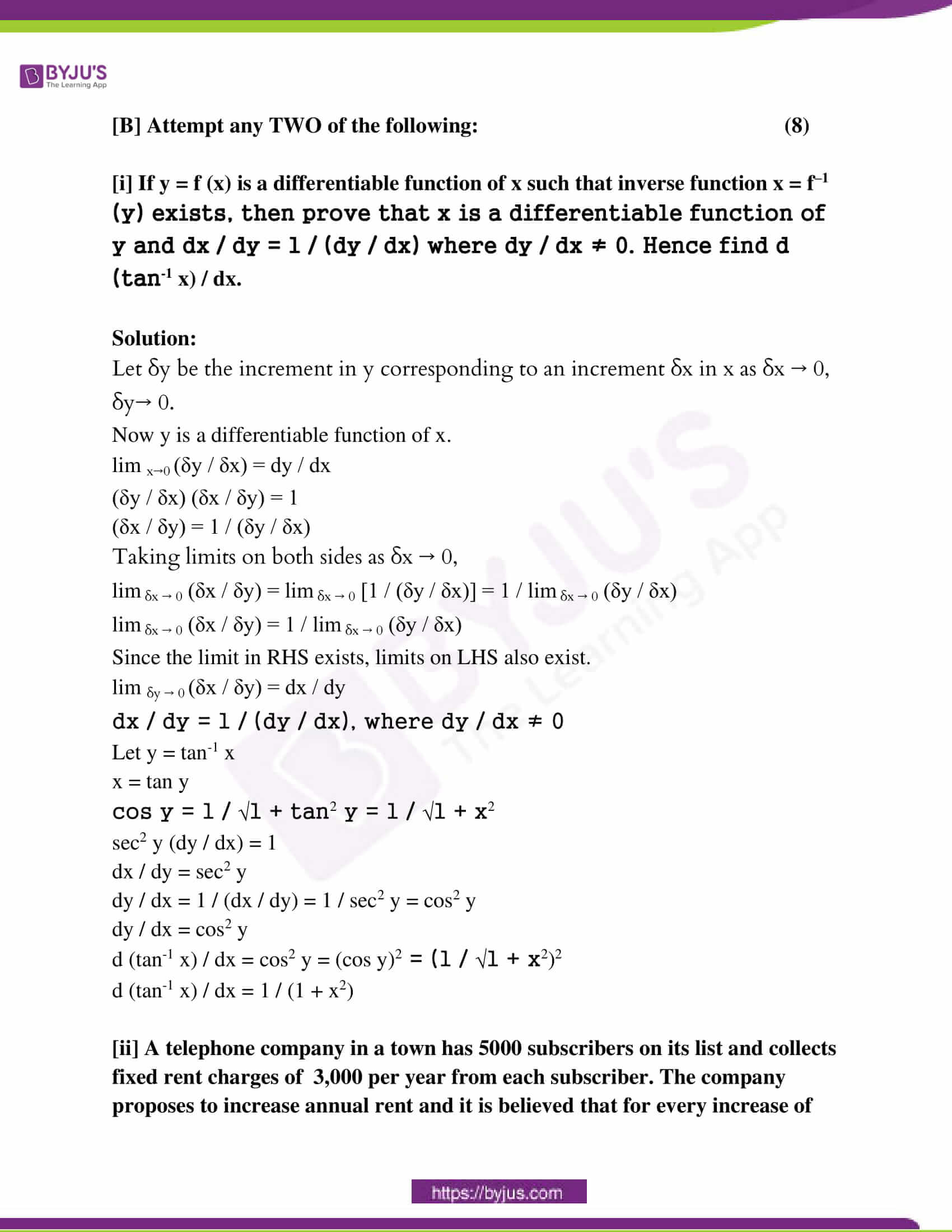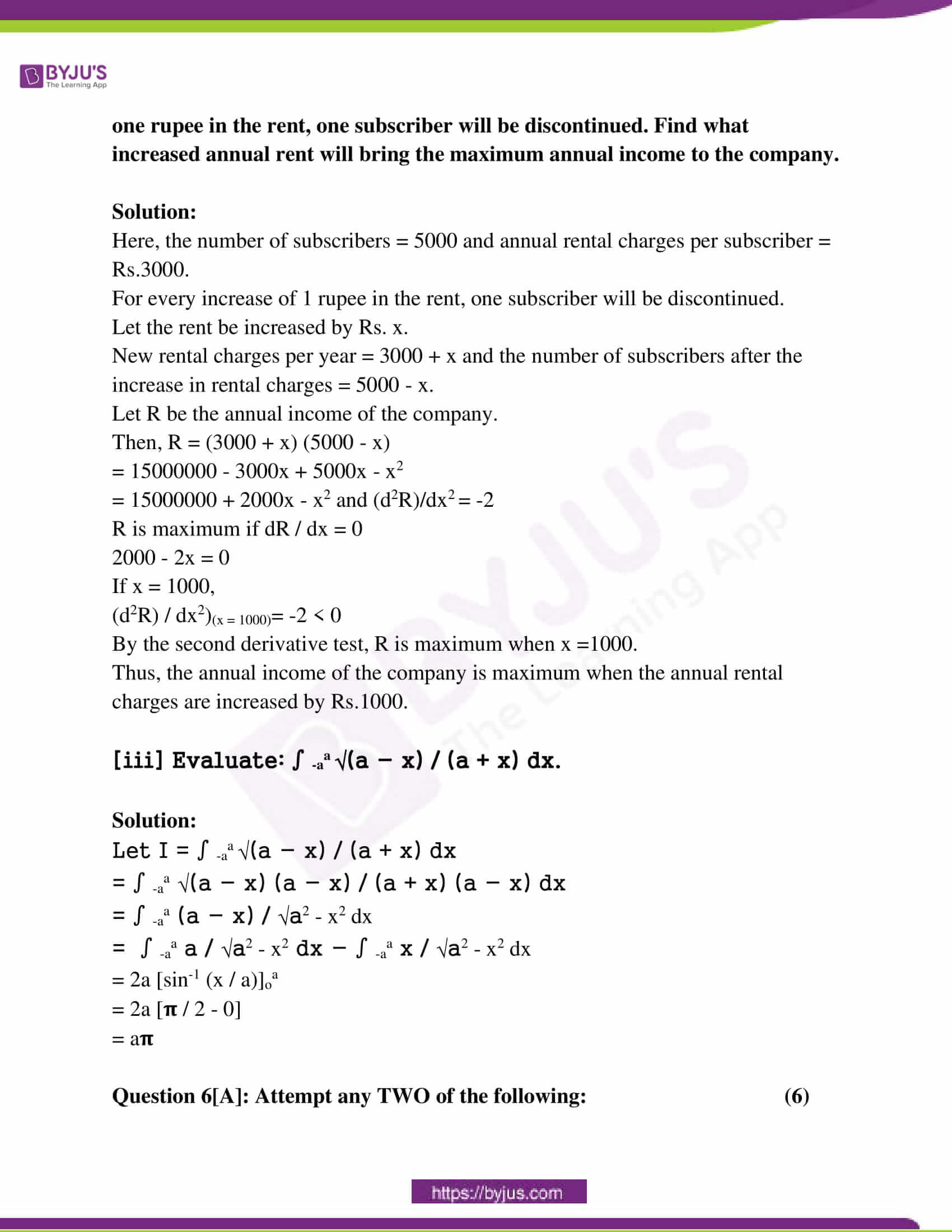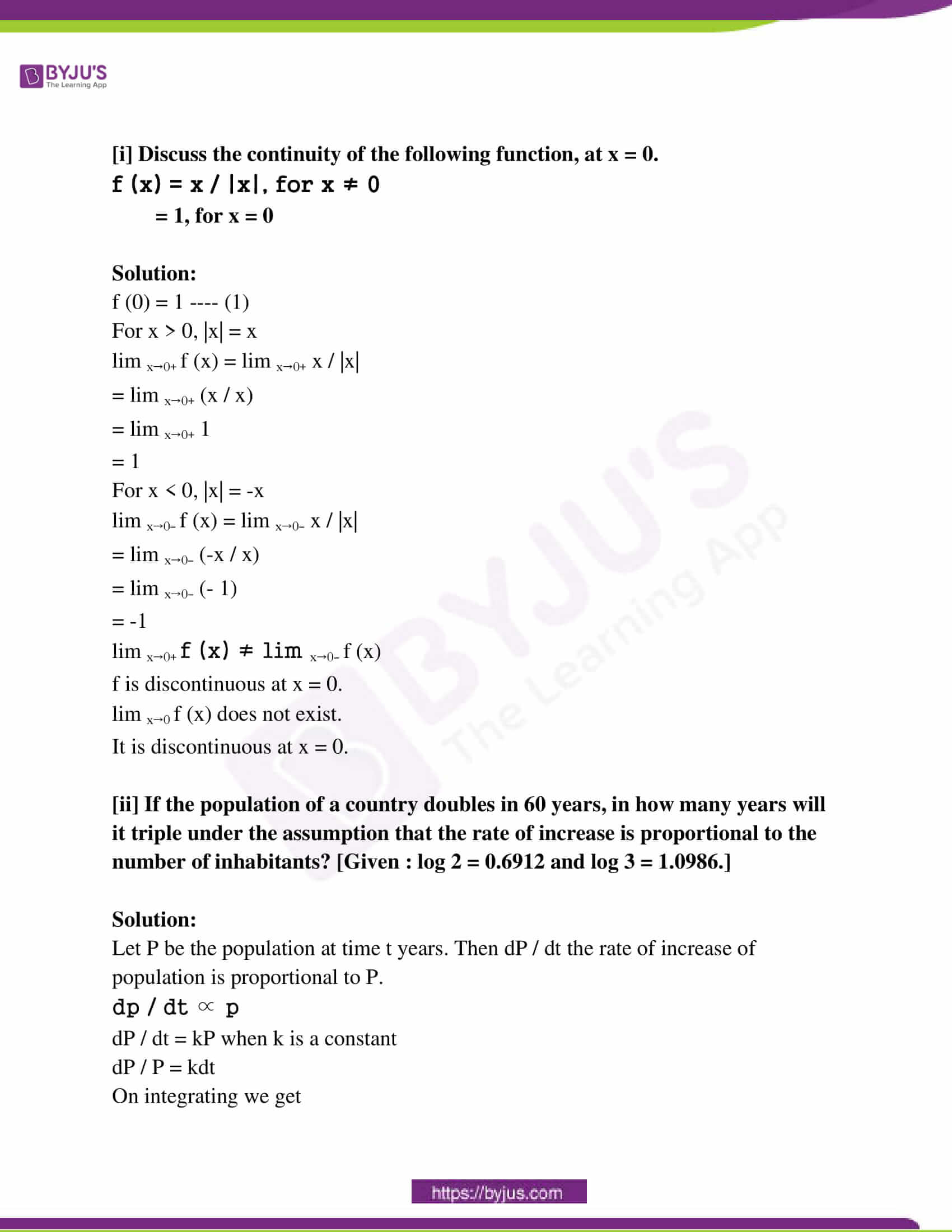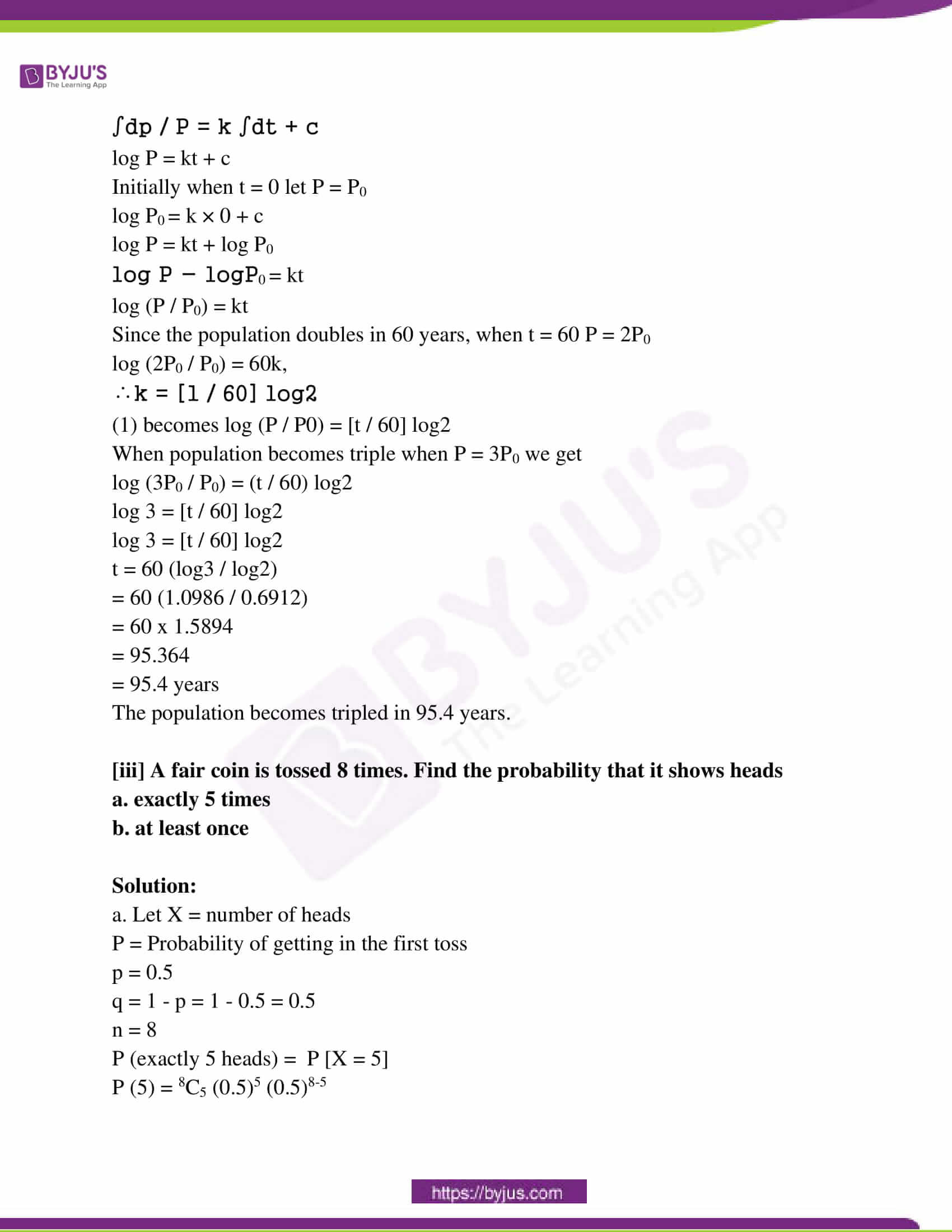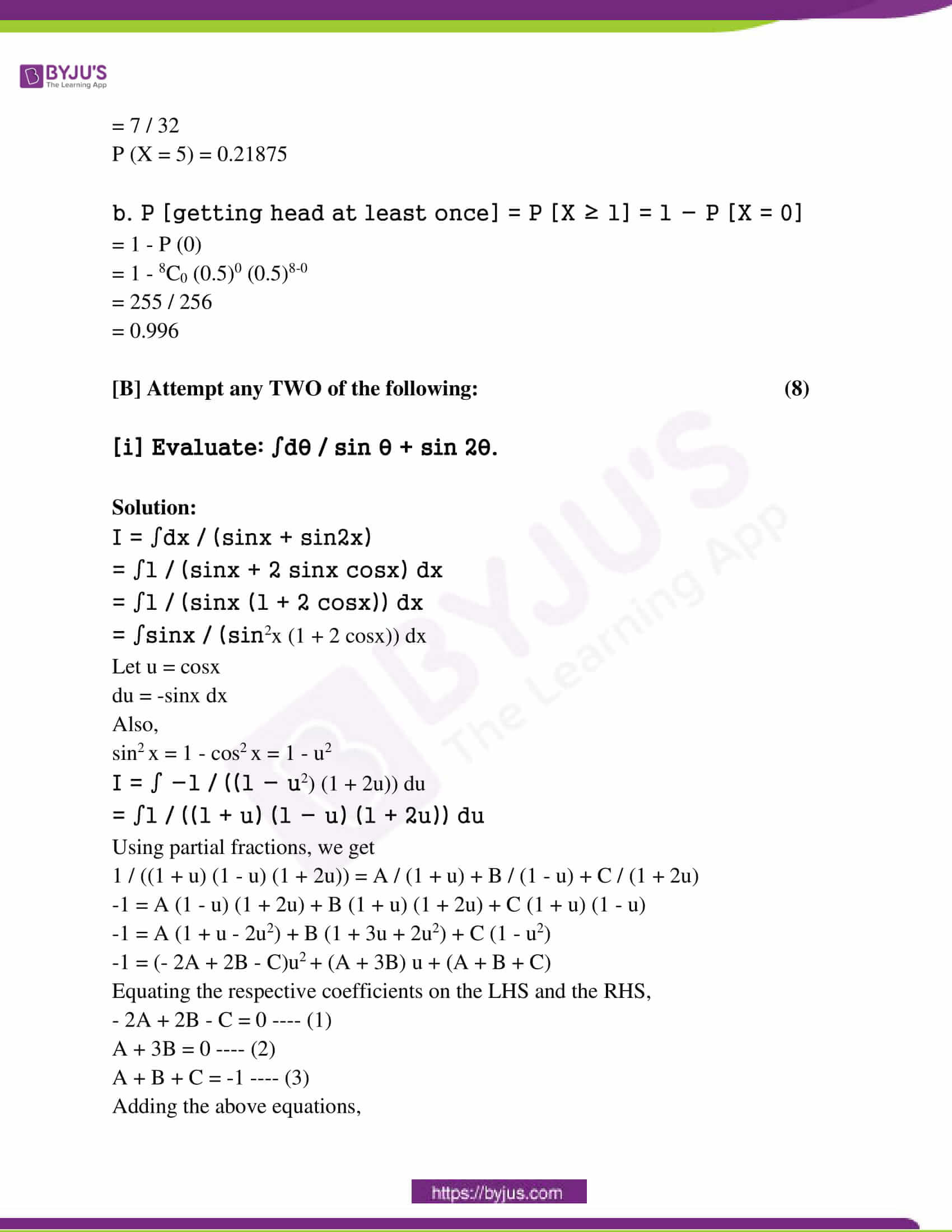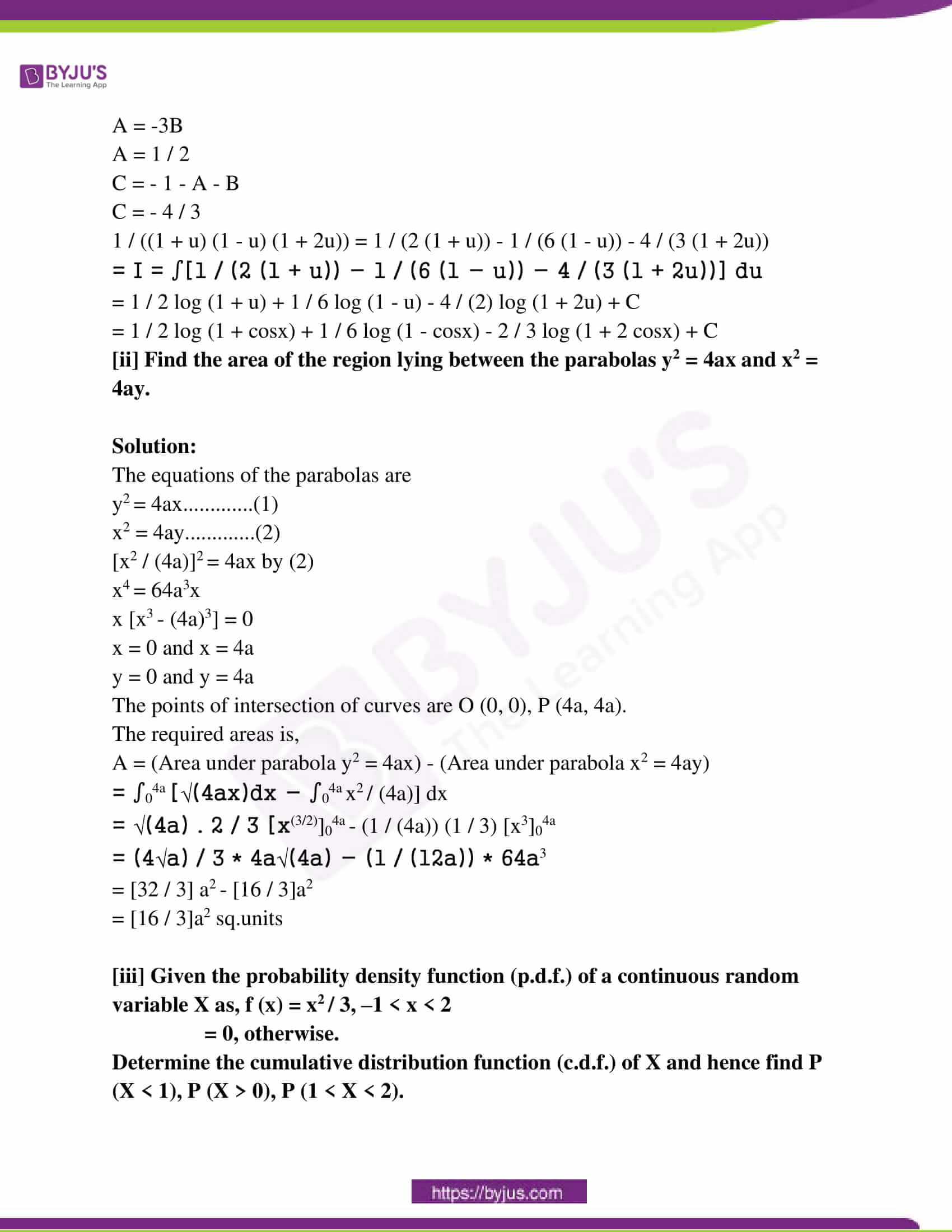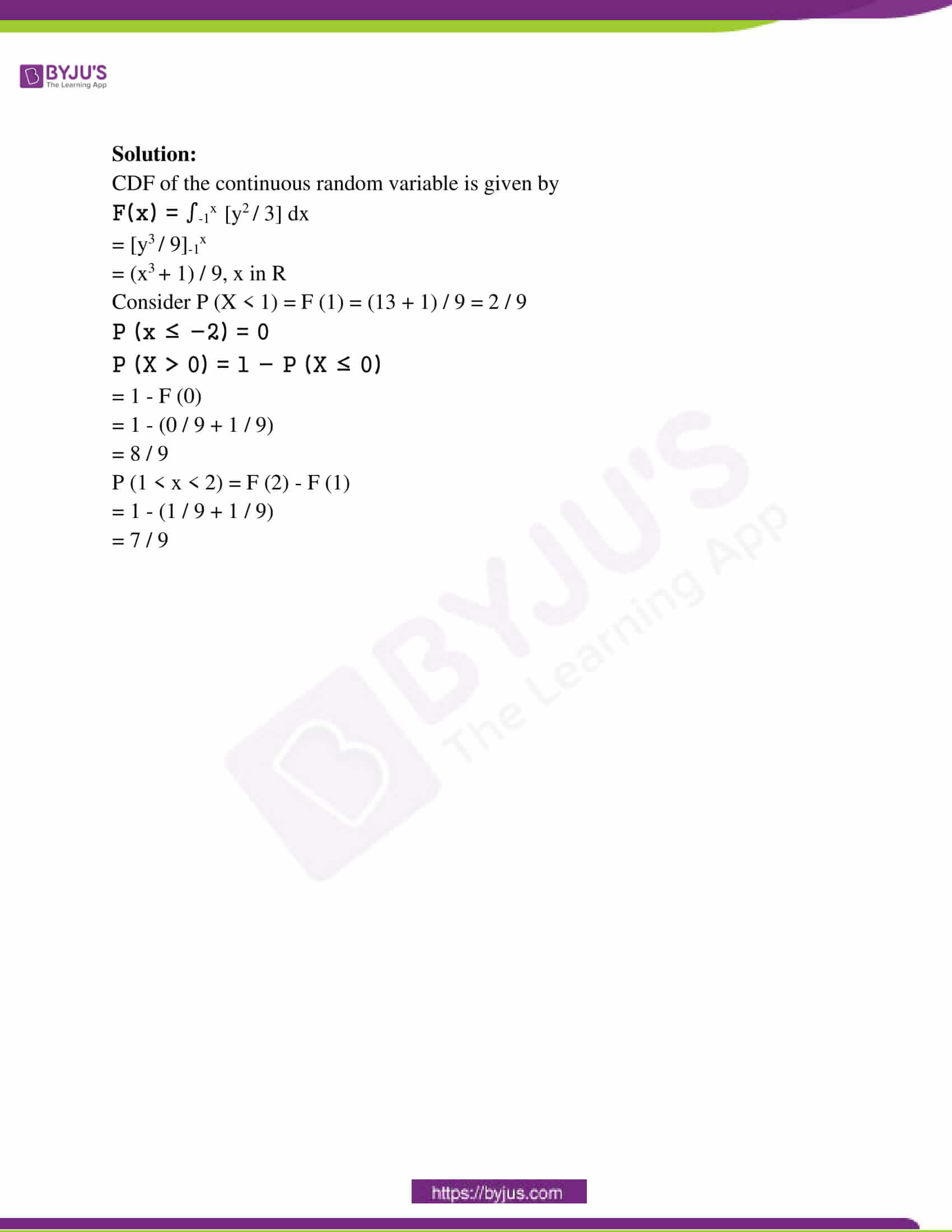SECTION – A

Question 1[A]: Select and write the most appropriate answer from the given alternatives in each of the following sub-questions. 

[i]: If the points A (2, 1, 1), B (0, 1, 4) and C (k, 3, 2) are collinear, then k =______.

(A) 0 (B) 1 (C) 4 (D) – 4

Direction ratio of AB = (-2, -2, 3)

Direction ratio of BC = (k, 4, -6)

If the points are collinear, then,

Direction ratio of AB / Direction ratio of BC = constant

-2 / k = -2 / 4

k = 4

[ii]: The inverse of matrix A =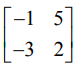is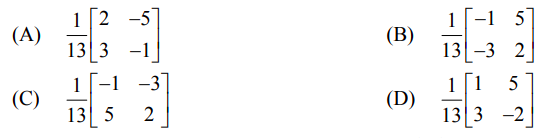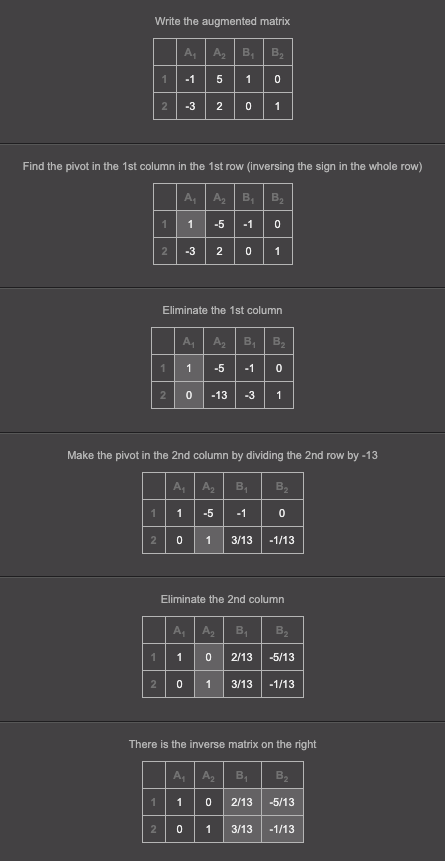[iii]: In △ABC, if a = 13, b = 14 and c = 15, then sin [A / 2] is

(A) 1 / 5 (B) √(1 / 5) (C) 4 / 5 (D) 2 / 5

a = 13, b = 14 and c = 15

s = [a + b + c] / 2

= [13 + 14 + 15] / 2

= 21

sin (A / 2) = √(s – b) (s – c) / b * c

= √(21 – 14) (21 – 15) / 14 * 15

= √7 * 6 / 14 * 15

= √1 / 5

[B] Attempt any THREE of the following: (6)

[i]: Find the volume of the parallele𝛑ped whose coterminous edges are given by vectors 2i + 3j – 4k, 5i + 7j + 5k and 4i + 5j – 2k.

Solution:

The volume of the parallele𝛑ped = [a b c]

a = 2i + 3j – 4k

b = 5i + 7j + 5k

c = 4i + 5j – 2k

V = [a b c]

= 2 (- 14 – 25) – 3( – 10 – 20) – 4 (25 – 28)

= 2 (- 39) – 3 (- 30) – 4 (- 3)

= – 78 + 90 + 12

= 24 cubic units

[ii]: In △ABC, prove that, a (b cos C – c cos B) = b2 – c2.

Solution:

Law of cosines: c² = a² + b² – 2ab cos y

b2 = a2 + c2 – 2ac cos B

c2 = a2 + b2 – 2ab cos C

The second equation is subtracted from the first one,

b2 – c2 = c2 – b2 – 2ac cos B + 2ab cos C

2b2 – 2c2 = – 2ac cos B + 2ab cos C

2 (b2 – c2) = 2a (- c cos B + b cos C)

b2 – c2 = a (b cos C – c cos B)

[iii]: If from a point Q (a, b, c) perpendiculars QA and QB are drawn to the YZ and ZX planes respectively, then find the vector equation of the plane QAB.

Solution:

QA and QB are the perpendiculars from the point Q( a, b, c) to YZ and ZX planes.

A = ( 0, b, c) and B = (a, 0, c)

The required plane is passing through O(0, 0, 0), A(0, b, c) and B(a, 0, c)

The vector equation of the plane passing through the O, A, B is

r . (OA x OB) = 0 . (OA x OB)

r . (a x b) = 0

OA = a = 0i + bj + ck

OB = b = ai + 0j + ck

OA x OB =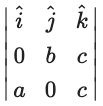= (bc – 0)i – (0 – ac)j + (0 – ab)k

= bci + acj – abk

The vector equation of the required plane is r . (bci + acj – abk) = 0.

[iv]: Find the cartesian equation of the line passing through the points A(3, 4, – 7) and B(6, – 1, 1).

Solution:

The cartesian equation of the line passing through the points A (x1, y1, z1) and B (x2, y2, z2) is

(x – x1) / (x2 – x1) = (y – y1) / (y2 – y1) = (z – z1) / (z2 – z1)

The cartesian equation of the line passing through the points A (3, 4 , – 7) and B (6, – 1, 1) is

(x – 3) / (6 – 3) = (y – 4) / (- 1 – 4) = (z – ( – 7)) / (1 – ( – 7))

(x – 3) / (3) = (y – 4) / (- 5) = (z + 7) / (8)

[v]: Write the following statement in symbolic form and find its truth value: ∀ n ε N, n2 + n is an even number and n2 – n is an odd number.

Solution:

Let p ≣ ∀ n ε N, n2 + n is an even number

Let q ≣ ∀ n ε N, n2 – n is an odd number

The symbolic form of the given statement is (p ∧ q)

The truth value of a given statement is

p ≣ ∀ n ε N, n2 + n is an even number (T)

q ≣ ∀ n ε N, n2 – n is an odd number (F)

(∵ from n = 1, n2 – n = 0, which is not an odd number)

(p ∧ q) ≣ T ∧ F ≣ F

So, the given statement is false.

Question 2[A]: Attempt any TWO of the following: (6)

[i] Using truth tables, examine whether the statement pattern (p ∧ q) ∨ (p ∧ r) is a tautology, contradiction or contingency.

Solution:

Number of rows = 2n = 23 = 8

Number of columns = m + n = 3 + 3 = 6

 p q r p ∧ q p ∧ r (p ∧ q) ∨ (p ∧ r) T T T T T T T T F T F T T F T F T T T F F F F F F T T F F F F T F F F F F F T F F F F F F F F F

Therefore, it is a contingency.

[ii] Find the shortest distance between the lines: x – 1 / 2 = y – 2 / 3 = z – 3 / 4 and x – 2 / 3 = y – 4 / 4 = z – 5 / 5.

Solution:

The lines are x – 1 / 2 = y – 2 / 3 = z – 3 / 4 —- (1)

x – 2 / 3 = y – 4 / 4 = z – 5 / 5 —- (2)

Here x1 = 1, y1 = 2, z1 = 3 and a1 = 3, b1 = 3, c1 = 4

x2 = 2, y2 = 4, z2 = 5 and a2 = 3, b2 = 4, c2 = 5

Shortest distance between two lines is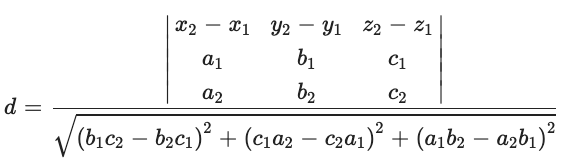= 1 (15 – 16) – 2 (10 – 12) + 2 (8 – 9)

= – 1 + 4 – 2

= 1

Denominator = (15 – 16)2 + (12 – 10)2 + (8 – 9)2 = 1 + 4 + 1 = 6

d = 1 / √6 units

[iii] Find the general solution of the equation sin 2x + sin 4x + sin 6x = 0.

Solution:

(sin 2x + sin 6x) + sin 4x =0

2sin4x . cos2x + sin4x = 0

sin 4x (2cos 2x + 1) = 0

sin 4x = 0 or 2 cos2x + 1 = 0

sin 4x = 0 or cos 2x = -1 / 2

= -cos 𝛑 / 3

= cos (𝛑 – 𝛑 / 3)

Using sinx = 0 ⇛ x = n𝛑

sin 4x = 0

4x = n𝛑

The general solution is x = (n𝛑) / 4

Using cosx = cosɑ ⇛ x = 2mx ± ɑ

cos 2x = cos ((2𝛑) / 3)

2x = 2m𝛑 ± (2𝛑) / 3

The general solution is

x = m𝛑 ± 𝛑 / 3 where m, n is in z.

[B] Attempt any TWO of the following: (8)

[i] Solve the following equations by method of reduction: x – y + z = 4, 2x + y – 3z = 0, x + y + z = 2.

Solution:

x – y + z = 4

2x + y – 3z = 0

x + y + z = 2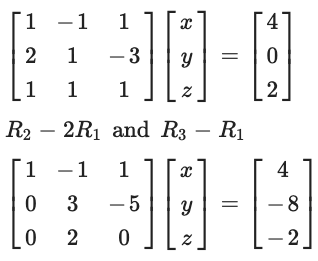x – y + z = 4 ….(1)

3y – 5z = – 8 ….(2)

2y = – 2 ….(3)

y = – 1

By equation (2)

-3 – 5z = – 8 – 5z = – 5z = 1

By equation (1)

x + 1 + 1 = 4

x = 2

x = 2, y = -1, z = 1

[ii] If θ is the measure of the acute angle between the lines represented by the equation ax2 + 2hxy + by2 = 0, then prove that tan θ = |2√h2 – ab / a + b| where a + b ≠ 0 and b ≠ 0. Find the condition for coincident lines.

Solution:

Let m1 and m2 be the slopes of the lines represented by the equation,

ax2 + 2hxy + by2 = 0 —- (1)

y = m1x and y = m2x

The combined equation is (y – m1x) (y – m2x) = 0

m1m2x2 – (m1 + m2)xy + y2 = 0 — (2)

Since equations (1) and (2) represents the same two lines, on comparing the coefficients,

m1m2 / a = 1 / b = m1 + m2 / 2h

m1 + m2 = – 2h / b and m1m2 = a / b

(m1 – m2)2 = (m1 + m2)2 – 4m1m2

(m1 – m2)2 = (- 2h / b)2 – 4 (a / b)

= 4 (h2 – ab) / b2

The angle between them is given by

tan θ = |m1 – m2 / 1 + m1 – m2|

= |√(m1 – m2)2 / 1 + m1 – m2|

= |4 √(h2 – ab) / b2 / 1 + (a / b)|

= |2√h2 – ab / a + b|

tan θ = |2√h2 – ab / a + b|

[iii] Using the vector method, find incentre of the triangle whose vertices are P (0, 4, 0), Q (0, 0, 3) and R(0, 4, 3).

Solution:

Let p, q, r be the position vectors of vertices P, Q, R of triangle PQR, respectively.

p = 4j, q = 3k, r = 4j + 3k

PQ = q – p = 3k – 4j = – 4j + 3k

QR = r – q = 4j + 3k – 3k = 4j

RP = p – r = 4j – 4j – 3k = – 3k

Let x, y, z be the lengths of opposites of vertices P, Q, R, respectively.

x = |QR| = 4

y = |RP| = 3

z = |PQ| = 5

The incentre of the triangle = h = (xp + yq + zr) / x + y + z

= 4 (4j) + 3 (3k) + 5 (4j + 3k) / 4 + 3 + 5

= 16j + 9k + 20j + 15k / 12

= 3j + 2k

Question 3[A]: Attempt any TWO of the following: (6)

[i] Construct the switching circuit for the statement (p ∧ q) ∨ (~ p) ∨ (p ∧ ~ q).

Solution:

Let p = S1 is closed and q = S2 is closed. ~p = switch S1’ and ~q = S2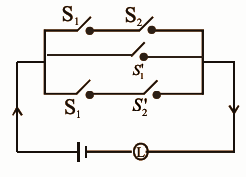[ii] Find the joint equation of the pair of lines passing through the origin which are perpendicular respectively to the lines represented by 5x2 + 2xy – 3y2 = 0.

Solution:

Given homogeneous equation is 5x2 + 2xy – 3y2 = 0

5x2 + 5xy – 3xy – 3y2 = 0

5x (x + y) – 3y (x + y ) = 0

(x + y) (5x – 3y) = 0

x + y = 0 and 5x – 3y = 0 are the two lines represented by the given equation.

The slopes are -1 and 5 / 3.

The required two lines are perpendicular to these lines.

The slopes of required lines are 1 and 3 / 5 and the lines pass through the origin.

The individual equations are

y = 1 . x and y = – 3 / 5 x

x – y = 0 and 3x + 5y = 0

Their joint equation is

(x – y) (3x + 5y) = 0

3x2 – 3xy + 5xy – 5y2 = 0

3x2 + 2xy – 5y2 = 0

[iii] Show that cos-1 (4 / 5) + cos-1 (12 / 13) = cos-1 (33 / 65).

Solution:

Let a = cos-1 (4 / 5) and b = cos-1 (12 / 13)

cos a = 4 / 5

sin2 a = 1 – cos2 a

sin a = √1 – cos2 a

= √1 – (4 / 5)2

= √1 – (16 / 25)

= √9 / 25

= 3 / 5

cos b = 12 / 13

sin2 b = 1 – cos2 b

sin b = √1 – cos2 b

= √1 – (12 / 13)2

= √1 – (144 / 169)

= √25 / 169

= 5 / 13

cos (a + b) = cos a cos b – sin a sin b

= (4 / 5) * (12 / 13) – (3 / 5) * (5 / 13)

= (48 – 15) / 65

= 33 / 65

cos (a + b) = 33 / 65

a + b = cos-1 (33 / 65)

cos-1 (4 / 5) + cos-1 (12 / 13) = cos-1 (33 / 65)

[B] Attempt any TWO of the following: (8)

[i] If l, m, n are the direction cosines of a line, then prove that l2 + m2 + n2 = 1. Hence find the direction angle of the line with the c-axis which makes direction angles of 135o and 45o with Y and Z axes, respectively.

Solution:

Let ɑ, β, 𝞬 be the angles made by the line with X, Y, Z axes, respectively.

l = cos ɑ, m = cos β and n = cos𝞬

Let a = a1i + a2j + a3k be any non-zero vector along the line.

Since i is the unit vector along X-axis,

a . i = |a| . |i| cos ɑ = a cosɑ

Also, a . i = (a1i + a2j + a3k). i

= a1 x 1 + a2 x 0 + a3 x 0 = a1

a cosɑ = a1 …………………………(1)

Since j is the unit vector along Y-axis,

a . j = |a| . |j| cosβ = a cosβ

a . j = (a1i + a2j + a3k) . j

= a1 x 0 + a2 x 1 + a3 x 0 = a2

a cos β = a2 ………………….(2)

a cos 𝞬 = a3 ………….(3)

From equations (1), (2) and (3),

a2 cos2 ɑ + a2 cos2 β + a2 cos2 𝞬 = a12 + a22 + a32

a2 (cos2 ɑ + cos2 β + cos2 𝞬) = a2 [a = |a| =√(a12 + a22 + a32)]

(cos2 ɑ + cos2 β + cos2 𝞬) = 1

l2 + m2 + n2 = 1

ɑ = ?, β = 135, 𝞬 = 45

(cos2 ɑ + cos2 β + cos2 𝞬) = 45

cos2 ɑ + cos2 135 + cos2 45 = 1

cos2 ɑ + 1 / 2 + 1 / 2 = 1

cosɑ = 0

ɑ = 𝛑 / 2 or (3𝛑) / 2

[ii] Find the vector and cartesian equations of the plane passing through the points A ( 1, 1, 2), B (1, 2, 1) and C (2, 1, 1).

Solution:

The vector equation of the plane passing through the points A (a), B (b) and C (c).

r . ((AB) x (AC)) = a ((AB) x (AC))…………(1)

Let a = i + j – 2k, b = i + 2j + k, c = 2i – j + k

(AB) = b – a = (i + 2j + k) – (i + j – 2k) = j + 3k and

(AC) = c – a = (2i – j + k) – (i – j – 2k) = i – 2j + 3k

(AB) x (AC) = |[i, j, k] , [0, 1, 3] , [1, -2, 3]|

= (3 + 6)i – (0 – 3)j + (0 – 1)k

= 9i + 3j – k

a . ((AB) x (AC)) = (i + j – 2k) . (9i + 3j – k)

= 1 (9) + 1 (3) + (-2) (-1)

= 9 + 3 + 2 = 14

From (1), the vector equation of the required plane is r . (9i + 3j – k) = 14

(xi + yj + zk) (9i + 3j- k) = 14

The cartesian equation of the plane is 9x + 3y – z = 14.

[iii] Solve the following L.P.P. by the graphical method:

Maximise : Z = 6x + 4y subject to x ≤ 2, x + y ≤ 3, -2x + y ≤ 1, x ≥ 0, y ≥ 0.

Solution: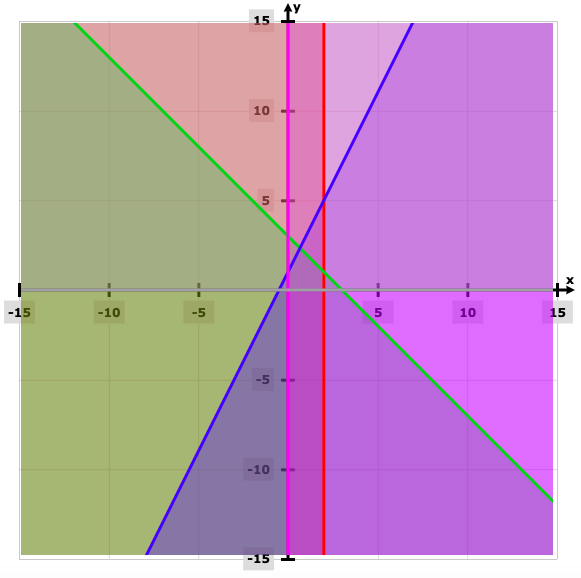Z = 6x + 4y

Z = 6x + 4y

Z at (0, 0) = 6 (0) + 4 (0) = 0

Z at (2, 0) = 6 (2) + 4 (0) = 12

Z at (2, 1) = 6 (2) + 4 (1) = 16

Z at (2 / 3, 7 / 3) = 6 (2 / 3) + (7 / 3) 4 = 40 / 3

Z at (0,1) = 6 (0) + 4 (1) = 4

Thus, Z is maximized at (2, 1) and its maximum value is 16.

SECTION – II

Question 4[A]: Select and write the appropriate answer from the given alternatives in each of the following sub-questions: (6)

[i] Derivatives of tan3 θ with respect to sec3 θ at θ = 𝛑 / 3 is _______.

(A) 3 / 2 (B) √3 / 2 (C) 1 / 2 (D) – √3 / 2

y = tan3 θ and x = sec3 θ

dy / dθ = 3 tan2 θ sec2 θ

dx / dθ = 3 sec2 θ sec θ tan θ

dy / dx = sin θ = sin (𝛑 / 3) = √3 / 2

[ii] The equation of tangent to the curve y = 3x2 – x + 1 at P(1, 3) is _______.

(A) 5x – y = 2 (B) x + 5y = 16 (C) 5x – y + 2 = 0 (D) 5x = y

dy / dx = 6x – 1 at (1, 3)

Slope of the tangent at (1, 3) = (6, 1) = 5

Equation of tangent is y – y1 = m (x – x1)

y – 3 = 5 (x – 1)

5x – y – 2 = 0

y = 5x – 2

[iii] The expected value of the number of heads obtained when three fair coins are tossed simultaneously is _______.

(A) 1 (B) 1.5 (C) 0 (D) 1

p = 1 / 2

q = 1 / 2

n = 3

E (X) = np = 3 * (0.5) = 1.5

[B] Attempt any THREE of the following: (6)

[i] Find dy / dx if x sin y + y sin x = 0.

Solution:

x sin y + y sin x = 0

[x cos y dy / dx + sin y] + [y cos x + sin x dy / dx] = 0

sin y + cos x = dy / dx [- sin x – x cos y]

dy / dx = [- sin x – x cos y] / [sin y + cos x]

[ii] Test whether the function, f (x) = x – (1 / x), x ∈ R, x ≠ 0, is increasing or decreasing.

Solution:

f (x) = x – (1 / x)

f ‘ (x) = 1 – (- 1 / x2) = 1 + (1 / x2)

x ≠ 0, for all values of x, x2 > 0

1 / x2 > 0, [1 + (1 / x2)] is always positive

Thus f ‘ (x) > 0, for all x ∈ R.

It is an increasing function.

[iii] Evaluate: ∫[sin √x / √x] dx.

Solution:

Let I = ∫[sin √x / √x] dx

Let √x = t

[1 / √x] dx = 2dt

I = 2 ∫sin t dt

= – 2 cos t + c

= – 2 cos √x + c

[iv] Form the differential equation by eliminating arbitrary constants from the relation y = Ae5x + Be-5x.

Solution:

y = Ae5x + Be-5x

On differentiating with respect to x,

dy / dx = Ae5x (5) + Be-5x (-5)

dy / dx = 5Ae5x – 5Be-5x

Again differentiating with respect to x,

d2y / dx2 = 5Ae5x (5) – 5Be-5x (-5)

d2y / dx2 = 25Ae5x + 25 Be-5x

= 25 [Ae5x + Be-5x]

= 25y

d2y / dx2 – 25y = 0

[v] The probability that a bomb will hit a target is 0.8. Find the probability that out of 10 bombs dropped, exactly 4 will hit the target.

Solution:

Let r be the number of bombs that hit the target.

p = 0.8

q = 1 – p = 1 = 0.8 = 0.2

n = 10

r = 4

P (r = 4) = nCr pr qn-r

= 10C4 (0.8)4 (0.2)10 – 4

= 210 * (2)18 * (1 / 10)10

= 55050240 / 1010

= anti (log 210 + 18 log 2 – 10)

= 0.0055

Question 5[A]: Attempt any TWO of the following: (6)

[i] Solve: dy / dx = cos (x + y).

Solution:

dy / dx = cos (x + y) —- (1)

Put x + y = v —- (2)

y = v – x

dy / dx = dv / dx – 1 —- (3)

Subtracting (2) and (3) in (1),

dv / dx – 1 = cos v

dv / dx = 1 + cos v

dv / dx = 2 cos2 (v / 2)

[1 / cos2 (v / 2)] dv = 2dx

sec2 (v / 2) dv = 2 dx

On integrating both sides,

∫sec2 (v / 2) dv = 2 ∫dx

2 tan (v / 2) = 2x + c

tan (v / 2) = x + (c / 2)

tan [(x + y) / 2] = x + c

[ii] If u and v are two functions of x, then prove that:

∫uv dx = u ∫v dx – ∫[du / dx ∫v dx] dx.

Solution:

Let ∫v dx = w —- (1)

dw / dx = v —- (2)

d (u, w) / dx = u . (d [w] / dx] + w . (d [u] / dx]

= u . v + w (du / dx) —- (from 2)

By the definition of integration,

u . w = ∫[u . v + w (du / dx)] dx

= ∫u . v dx + ∫w . (du / dx) dx

∫u . v dx = u . w – ∫w . (du / dx) dx

= u ∫v dx – ∫[du / dx ∫ v dx] dx

[iii] If f (x) =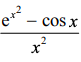, for x ≠ 0, is continuous at x = 0, find f (0).

Solution:

f (x) is continuous at x = 0.

lim x→0 f (x) = f (0)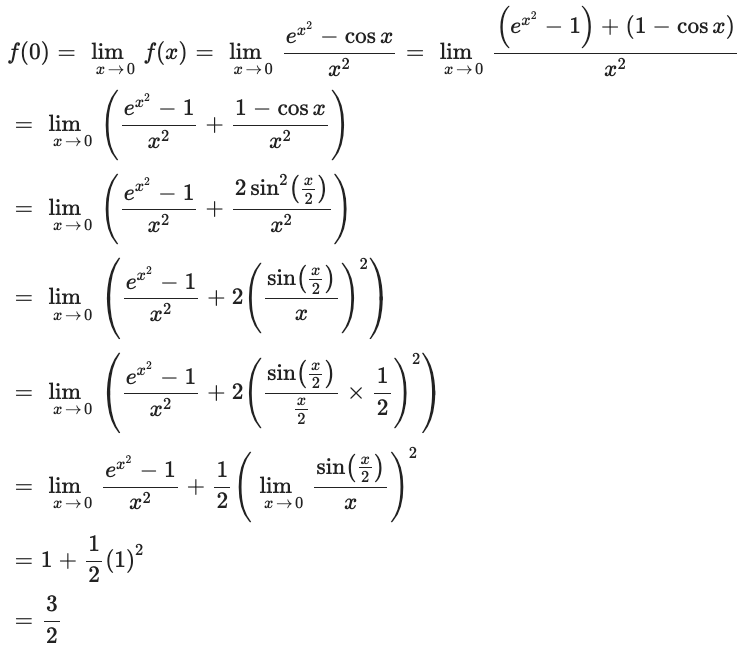[B] Attempt any TWO of the following: (8)

[i] If y = f (x) is a differentiable function of x such that inverse function x = f–1 (y) exists, then prove that x is a differentiable function of y and dx / dy = 1 / (dy / dx) where dy / dx ≠ 0. Hence find d (tan-1 x) / dx.

Solution:

Let δy be the increment in y corresponding to an increment δx in x as δx → 0, δy→ 0.

Now y is a differentiable function of x.

lim x→0 (δy / δx) = dy / dx

(δy / δx) (δx / δy) = 1

(δx / δy) = 1 / (δy / δx)

Taking limits on both sides as δx → 0,

lim δx → 0 (δx / δy) = lim δx → 0 [1 / (δy / δx)] = 1 / lim δx → 0 (δy / δx)

lim δx → 0 (δx / δy) = 1 / lim δx → 0 (δy / δx)

Since the limit in RHS exists, limits on LHS also exist.

lim δy → 0 (δx / δy) = dx / dy

dx / dy = 1 / (dy / dx), where dy / dx ≠ 0

Let y = tan-1 x

x = tan y

cos y = 1 / √1 + tan2 y = 1 / √1 + x2

sec2 y (dy / dx) = 1

dx / dy = sec2 y

dy / dx = 1 / (dx / dy) = 1 / sec2 y = cos2 y

dy / dx = cos2 y

d (tan-1 x) / dx = cos2 y = (cos y)2 = (1 / √1 + x2)2

d (tan-1 x) / dx = 1 / (1 + x2)

[ii] A telephone company in a town has 5000 subscribers on its list and collects fixed rent charges of 3,000 per year from each subscriber. The company proposes to increase annual rent and it is believed that for every increase of one rupee in the rent, one subscriber will be discontinued. Find what increased annual rent will bring the maximum annual income to the company.

Solution:

Here, the number of subscribers = 5000 and annual rental charges per subscriber = Rs.3000.

For every increase of 1 rupee in the rent, one subscriber will be discontinued.

Let the rent be increased by Rs. x.

New rental charges per year = 3000 + x and the number of subscribers after the increase in rental charges = 5000 – x.

Let R be the annual income of the company.

Then, R = (3000 + x) (5000 – x)

= 15000000 – 3000x + 5000x – x2

= 15000000 + 2000x – x2 and (d2R)/dx2 = -2

R is maximum if dR / dx = 0

2000 – 2x = 0

If x = 1000,

(d2R) / dx2)(x = 1000)= -2 < 0

By the second derivative test, R is maximum when x =1000.

Thus, the annual income of the company is maximum when the annual rental charges are increased by Rs.1000.

[iii] Evaluate: ∫ -aa √(a – x) / (a + x) dx.

Solution:

Let I = ∫ -aa √(a – x) / (a + x) dx

= ∫ -aa √(a – x) (a – x) / (a + x) (a – x) dx

= ∫ -aa (a – x) / √a2 – x2 dx

= ∫ -aa a / √a2 – x2 dx – ∫ -aa x / √a2 – x2 dx

= 2a [sin-1 (x / a)]oa

= 2a [𝛑 / 2 – 0]

= a𝛑

Question 6[A]: Attempt any TWO of the following: (6)

[i] Discuss the continuity of the following function, at x = 0.

f (x) = x / |x|, for x ≠ 0

= 1, for x = 0

Solution:

f (0) = 1 —- (1)

For x > 0, |x| = x

lim x→0+ f (x) = lim x→0+ x / |x|

= lim x→0+ (x / x)

= lim x→0+ 1

= 1

For x < 0, |x| = -x

lim x→0- f (x) = lim x→0- x / |x|

= lim x→0- (-x / x)

= lim x→0- (- 1)

= -1

lim x→0+ f (x) ≠ lim x→0- f (x)

f is discontinuous at x = 0.

lim x→0 f (x) does not exist.

It is discontinuous at x = 0.

[ii] If the population of a country doubles in 60 years, in how many years will it triple under the assumption that the rate of increase is proportional to the number of inhabitants? [Given : log 2 = 0.6912 and log 3 = 1.0986.]

Solution:

Let P be the population at time t years. Then dP / dt the rate of increase of population is proportional to P.

dp / dt ∝ p

dP / dt = kP when k is a constant

dP / P = kdt

On integrating we get

∫dp / P = k ∫dt + c

log P = kt + c

Initially when t = 0 let P = P0

log P0 = k × 0 + c

log P = kt + log P0

log P − logP0 = kt

log (P / P0) = kt

Since the population doubles in 60 years, when t = 60 P = 2P0

log (2P0 / P0) = 60k,

∴k = [1 / 60] log2

(1) becomes log (P / P0) = [t / 60] log2

When population becomes triple when P = 3P0 we get

log (3P0 / P0) = (t / 60) log2

log 3 = [t / 60] log2

log 3 = [t / 60] log2

t = 60 (log3 / log2)

= 60 (1.0986 / 0.6912)

= 60 x 1.5894

= 95.364

= 95.4 years

The population becomes tripled in 95.4 years.

[iii] A fair coin is tossed 8 times. Find the probability that it shows heads

a. exactly 5 times

b. at least once

Solution:

a. Let X = number of heads

P = Probability of getting in the first toss

p = 0.5

q = 1 – p = 1 – 0.5 = 0.5

n = 8

P (exactly 5 heads) = P [X = 5]

P (5) = 8C5 (0.5)5 (0.5)8-5

= 7 / 32

P (X = 5) = 0.21875

b. P [getting head at least once] = P [X ≥ 1] = 1 – P [X = 0]

= 1 – P (0)

= 1 – 8C0 (0.5)0 (0.5)8-0

= 255 / 256

= 0.996

[B] Attempt any TWO of the following: (8)

[i] Evaluate: ∫dθ / sin θ + sin 2θ.

Solution:

I = ∫dx / (sinx + sin2x)

= ∫1 / (sinx + 2 sinx cosx) dx

= ∫1 / (sinx (1 + 2 cosx)) dx

= ∫sinx / (sin2x (1 + 2 cosx)) dx

Let u = cosx

du = -sinx dx

Also,

sin2 x = 1 – cos2 x = 1 – u2

I = ∫ -1 / ((1 – u2) (1 + 2u)) du

= ∫1 / ((1 + u) (1 – u) (1 + 2u)) du

Using partial fractions, we get

1 / ((1 + u) (1 – u) (1 + 2u)) = A / (1 + u) + B / (1 – u) + C / (1 + 2u)

-1 = A (1 – u) (1 + 2u) + B (1 + u) (1 + 2u) + C (1 + u) (1 – u)

-1 = A (1 + u – 2u2) + B (1 + 3u + 2u2) + C (1 – u2)

-1 = (- 2A + 2B – C)u2 + (A + 3B) u + (A + B + C)

Equating the respective coefficients on the LHS and the RHS,

– 2A + 2B – C = 0 —- (1)

A + 3B = 0 —- (2)

A + B + C = -1 —- (3)

A = -3B

A = 1 / 2

C = – 1 – A – B

C = – 4 / 3

1 / ((1 + u) (1 – u) (1 + 2u)) = 1 / (2 (1 + u)) – 1 / (6 (1 – u)) – 4 / (3 (1 + 2u))

= I = ∫[1 / (2 (1 + u)) – 1 / (6 (1 – u)) – 4 / (3 (1 + 2u))] du

= 1 / 2 log (1 + u) + 1 / 6 log (1 – u) – 4 / (2) log (1 + 2u) + C

= 1 / 2 log (1 + cosx) + 1 / 6 log (1 – cosx) – 2 / 3 log (1 + 2 cosx) + C

[ii] Find the area of the region lying between the parabolas y2 = 4ax and x2 = 4ay.

Solution:

The equations of the parabolas are

y2 = 4ax………….(1)

x2 = 4ay………….(2)

[x2 / (4a)]2 = 4ax by (2)

x4 = 64a3x

x [x3 – (4a)3] = 0

x = 0 and x = 4a

y = 0 and y = 4a

The points of intersection of curves are O (0, 0), P (4a, 4a).

The required areas is,

A = (Area under parabola y2 = 4ax) – (Area under parabola x2 = 4ay)

= ∫04a [√(4ax)dx – ∫04a x2 / (4a)] dx

= √(4a) . 2 / 3 [x(3/2)]04a – (1 / (4a)) (1 / 3) [x3]04a

= (4√a) / 3 * 4a√(4a) – (1 / (12a)) * 64a3

= [32 / 3] a2 – [16 / 3]a2

= [16 / 3]a2 sq.units

[iii] Given the probability density function (p.d.f.) of a continuous random variable X as, f (x) = x2 / 3, –1 < x < 2

= 0, otherwise.

Determine the cumulative distribution function (c.d.f.) of X and hence find P (X < 1), P (X > 0), P (1 < X < 2).

Solution:

CDF of the continuous random variable is given by

F(x) = ∫-1x [y2 / 3] dx

= [y3 / 9]-1x

= (x3 + 1) / 9, x in R

Consider P (X < 1) = F (1) = (13 + 1) / 9 = 2 / 9

P (x ≤ -2) = 0

P (X > 0) = 1 – P (X ≤ 0)

= 1 – F (0)

= 1 – (0 / 9 + 1 / 9)

= 8 / 9

P (1 < x < 2) = F (2) – F (1)

= 1 – (1 / 9 + 1 / 9)

= 7 / 9